• 1. 问题引入——函数在一点连续的定义及几何意义 2. 函数一致连续的定义 ...4. 一致连续的几何解释（例：正弦函数的一致连续性） 5. 康托尔定理、聚点原理、函数在开区间内一致连续的充要条件 ...

1. 问题引入——函数在一点连续的定义及几何意义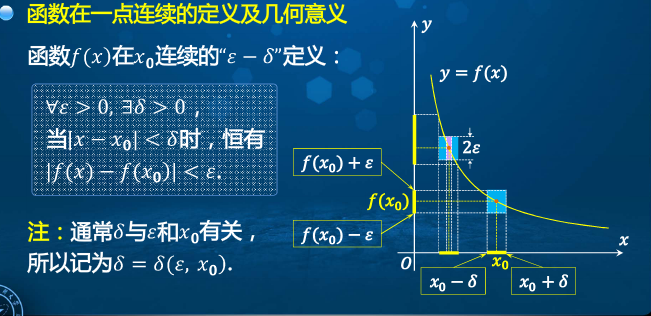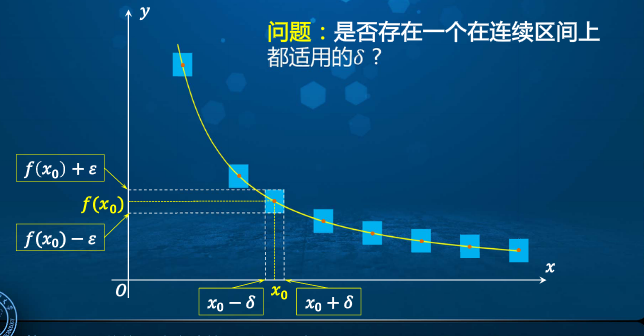2. 函数一致连续的定义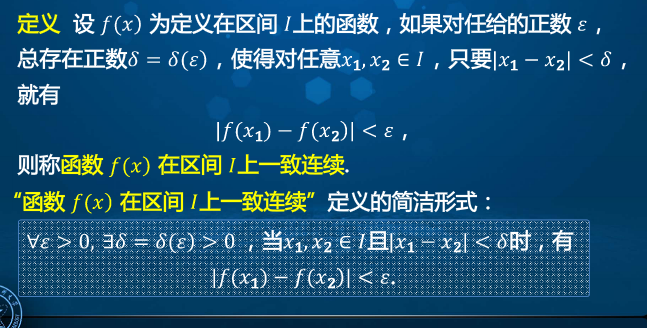3. 一致连续与连续的关系：若函数在开区间内一致连续，则其在该区间内连续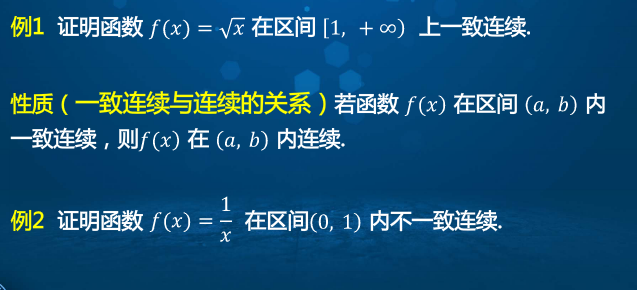4. 一致连续的几何解释（例：正弦函数的一致连续性）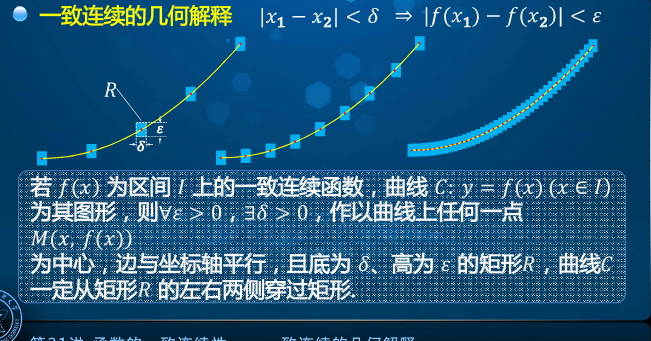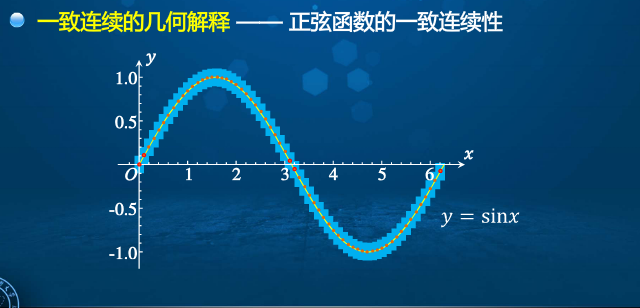5. 康托尔定理、聚点原理、函数在开区间内一致连续的充要条件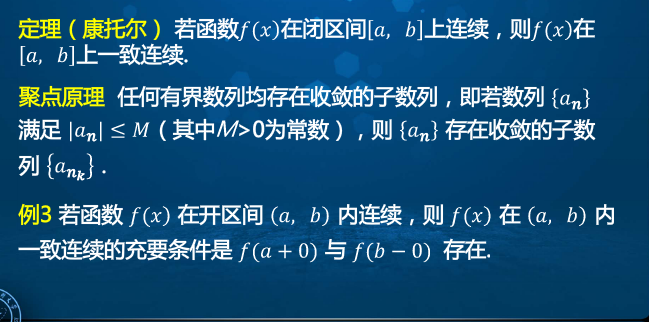展开全文• 利用Lyapunov 有 限时间稳定理论和矩阵理论, 给出了这类算法使得系统能够在有限时间内达到一致充分条件, 进而给出了一个 满足条件的有限时间一致性算法, 并对该算法的收敛进行分析, 得到了系统的收敛...
• 研究时变时滞与切换有... 假定出现的每一个通信拓扑都是可一致的, 借助时滞切换系统稳定的平均驻留时间方法, 以线性矩阵不等式(LMIs) 形式给出多智能体系统达到全局一致充分条件. 数值实例验证了结果的正确.</p>
• 本文关注具有固定拓扑和时变延迟的连续时间二阶... 事实证明，异步一致等效于具有时变的时变离散时间系统的全局渐近稳定。 此外，借助李雅普诺夫的直接方法，为异步共识建立了充分条件。 进行仿真以验证理论结果。
• ## 浅析数据一致性

万次阅读 多人点赞 2016-02-19 15:27:38
什么是数据一致性?  在数据有多分副本的情况下，如果网络、服务器或者软件出现故障，会导致部分副本写入成功，部分副本写入失败。这就造成各个副本之间的数据不一致，数据内容冲突。 实践中，导致数据不一致的情况...

欢迎支持笔者新作：《深入理解Kafka:核心设计与实践原理》和《RabbitMQ实战指南》，同时欢迎关注笔者的微信公众号：朱小厮的博客。什么是数据一致性?
在数据有多分副本的情况下，如果网络、服务器或者软件出现故障，会导致部分副本写入成功，部分副本写入失败。这就造成各个副本之间的数据不一致，数据内容冲突。 实践中，导致数据不一致的情况有很多种，表现样式也多种多样，比如数据更新返回操作失败，事实上数据在存储服务器已经更新成功。

CAP定理
CAP定理是2000年，由 Eric Brewer 提出来的。Brewer认为在分布式的环境下设计和部署系统时，有3个核心的需求，以一种特殊的关系存在。这里的分布式系统说的是在物理上分布的系统，比如我们常见的web系统。   这3个核心的需求是：Consistency，Availability和Partition Tolerance，赋予了该理论另外一个名字 － CAP。   Consistency：一致性，这个和数据库ACID的一致性类似，但这里关注的所有数据节点上的数据一致性和正确性，而数据库的ACID关注的是在在一个事务内，对数据的一些约束。系统在执行过某项操作后仍然处于一致的状态。在分布式系统中，更新操作执行成功后所有的用户都应该读取到最新值。   Availability：可用性，每一个操作总是能够在一定时间内返回结果。需要注意“一定时间”和“返回结果”。“一定时间”是指，系统结果必须在给定时间内返回。“返回结果”是指系统返回操作成功或失败的结果。   Partition Tolerance：分区容忍性，是否可以对数据进行分区。这是考虑到性能和可伸缩性。   CAP定理认为，一个提供数据服务的存储系统无法同事满足数据一致性、数据可用性、分区容忍性。   为什么不能完全保证这个三点了，个人觉得主要是因为一旦进行分区了，就说明了必须节点之间必须进行通信，涉及到通信，就无法确保在有限的时间内完成指定的行文，如果要求两个操作之间要完整的进行，因为涉及到通信，肯定存在某一个时刻只完成一部分的业务操作，在通信完成的这一段时间内，数据就是不一致性的。如果要求保证一致性，那么就必须在通信完成这一段时间内保护数据，使得任何访问这些数据的操作不可用。   如果想保证一致性和可用性，那么数据就不能够分区。一个简单的理解就是所有的数据就必须存放在一个数据库里面，不能进行数据库拆分。这个对于大数据量，高并发的互联网应用来说，是不可接受的。   在大型网站应用中，数据规模总是快速扩张的，因此可伸缩性即分区容忍性必不可少，规模变大以后，机器数量也会变得庞大，这是网络和服务器故障会频繁出现，要想保证应用可用，就必须保证分布式处理系统的高可用性。所以在大型网站中，通常会选择强化分布式存储系统的可用性(A)和伸缩性§，在某种程度上放弃一致性©。一般来说，数据不一致通常出现在系统高并发写操作或者集群状态不稳（故障恢复、集群扩容等）的情况下，应用系统需要对分布式数据处理系统的数据不一致性有所了解并进行某种意义上的补偿和纠错，以避免出现应用系统数据不正确。

数据一致性模型
一些分布式系统通过复制数据来提高系统的可靠性和容错性，并且将数据的不同的副本存放在不同的机器，由于维护数据副本的一致性代价高，因此许多系统采用弱一致性来提高性能，一些不同的一致性模型也相继被提出。
强一致性： 要求无论更新操作实在哪一个副本执行，之后所有的读操作都要能获得最新的数据。弱一致性：用户读到某一操作对系统特定数据的更新需要一段时间，我们称这段时间为“不一致性窗口”。最终一致性：是弱一致性的一种特例，保证用户最终能够读取到某操作对系统特定数据的更新。

数据一致性实现技术
Quorum系统NRW策略
这个协议有三个关键字N、R、W。
N代表数据所具有的副本数。R表示完成读操作所需要读取的最小副本数，即一次读操作所需要参与的最小节点数目。W表示完成写操作所需要写入的最小副本数，即一次写操作所需要参与的最小节点数目。
该策略中，只需要保证R+W>N，就可以保证强一致性。   例如：N=3，W=2，R=2，那么表示系统中数据有3个不同的副本，当进行写操作时，需要等待至少有2个副本完成了该写操作系统才会返回执行成功的状态，对于读操作，系统有同样的特性。由于R + W > N，因此该系统是可以保证强一致性的。   R + W> N会产生类似Quorum的效果。该模型中的读（写）延迟由最慢的R(W)副本决定，有时为了获得较高的性能和较小的延迟，R和W的和可能小于N，这时系统不能保证读操作能获取最新的数据。   如果R + W > N，那么分布式系统就会提供强一致性的保证，因为读取数据的节点和被同步写入的节点是有重叠的。在关系型数据管理系统中，如果N=2，可以设置为W=2，R=1，这是比较强的一致性约束，写操作的性能比较低，因为系统需要2个节点上的数据都完成更新后才将确认结果返回给用户。   如果R + W ≤ N，这时读取和写入操作是不重叠的，系统只能保证最终一致性，而副本达到一致的时间则依赖于系统异步更新的实现方式，不一致性的时间段也就等于从更新开始到所有的节点都异步完成更新之间的时间。 R和W的设置直接影响系统的性能、扩展性与一致性。如果W设置为1，则一个副本完成更改就可以返回给用户，然后通过异步的机制更新剩余的N-W的副本；如果R设置为1，只要有一个副本被读取就可以完成读操作，R和W的值如较小会影响一致性，较大则会影响性能，因此对这两个值的设置需要权衡。

下面为不同设置的几种特殊情况: 1. 当W=1,R=N时，系统对写操作有较高的要求，但读操作会比较慢，若N个节点中有节点发生故障，那么读操作将不能完成。 2. 当R=1,W=N时，系统对读操作有较高性能、高可用，但写操作性能较低，用于需要大量读操作的系统，若N个节点中有节点发生故障，那么些操作将不能完成。 3. 当R=Q,W=Q(Q=N/2+1)时，系统在读写性能之间取得平衡，兼顾了性能和可用性。

两阶段提交算法
在两阶段提交协议中，系统一般包含两类机器（或节点）：一类为协调者（coordinator），通常一个系统中只有一个；另一类为事务参与者（participants，cohorts或workers），一般包含多个，在数据存储系统中可以理解为数据副本的个数。两阶段提交协议由两个阶段组成，在正常的执行下，这两个阶段的执行过程如下所述：
阶段1：请求阶段（commit-request phase，或称表决阶段，voting phase）。 在请求阶段，协调者将通知事务参与者准备提交或取消事务，然后进入表决过程。在表决过程中，参与者将告知协调者自己的决策：同意（事务参与者本地作业执行成功）或取消（本地作业执行故障）。阶段2：提交阶段（commit phase）。 在该阶段，协调者将基于第一个阶段的投票结果进行决策：提交或取消。当且仅当所有的参与者同意提交事务协调者才通知所有的参与者提交事务，否则协调者将通知所有的参与者取消事务。参与者在接收到协调者发来的消息后将执行响应的操作。
举个例子：A组织B、C和D三个人去爬长城：如果所有人都同意去爬长城，那么活动将举行；如果有一人不同意去爬长城，那么活动将取消。用2PC算法解决该问题的过程如下：
首先A将成为该活动的协调者，B、C和D将成为该活动的参与者。阶段1：A发邮件给B、C和D，提出下周三去爬山，问是否同意。那么此时A需要等待B、C和D的邮件。B、C和D分别查看自己的日程安排表。B、C发现自己在当日没有活动安排，则发邮件告诉A它们同意下周三去爬长城。由于某种原因，D白天没有查看邮件。那么此时A、B和C均需要等待。到晚上的时候，D发现了A的邮件，然后查看日程安排，发现周三当天已经有别的安排，那么D回复A说活动取消吧。阶段2：此时A收到了所有活动参与者的邮件，并且A发现D下周三不能去爬山。那么A将发邮件通知B、C和D，下周三爬长城活动取消。此时B、C回复A“太可惜了”，D回复A“不好意思”。至此该事务终止。
两阶段提交算法在分布式系统结合，可实现单用户对文件（对象）多个副本的修改，多副本数据的同步。其结合的原理如下：
客户端（协调者）向所有的数据副本的存储主机（参与者）发送：修改具体的文件名、偏移量、数据和长度信息，请求修改数据，该消息是1阶段的请求消息。存储主机接收到请求后，备份修改前的数据以备回滚，修改文件数据后，向客户端回应修改成功的消息。如果存储主机由于某些原因（磁盘损坏、空间不足等）不能修改数据，回应修改失败的消息。客户端接收发送出去的每一个消息回应，如果存储主机全部回应都修改成功，向每存储主机发送确认修改的提交消息；如果存在存储主机回应修改失败，或者超时未回应，客户端向所有存储主机发送取消修改的提交消息。该消息是2阶段的提交消息。存储主机接收到客户端的提交消息，如果是确认修改，则直接回应该提交OK消息；如果是取消修改，则将修改数据还原为修改前，然后回应取消修改OK的消息。客户端接收全部存储主机的回应，整个操作成功。
在该过程中可能存在通信失败，例如网络中断、主机宕机等诸多的原因，对于未在算法中定义的其它异常，都认为是提交失败，都需要回滚，这是该算法基于确定的通信回复实现的，在参与者的确定回复（无论是回复失败还是回复成功）之上执行逻辑处理，符合确定性的条件当然能够获得确定性的结果哲学原理。   缺点：单个A是个严重问题：没有热备机制，A节点宕机了或者链接它的网络坏了会阻塞该事务；吞吐量不行，没有充分发动更多A的力量，一旦某个A第一阶段投了赞成票就得在它上面加独占锁，其他事务不得接入，直到当前事务提交or回滚。
分布式锁服务
分布式锁是对数据被外界修改持保守态度，在整个数据处理过程中将数据处于锁定状态，在用户修改数据的同时，其它用户不允许修改。   采用分布式锁服务实现数据一致性，是在操作目标之前先获取操作许可，然后再执行操作，如果其他用户同时尝试操作该目标将被阻止，直到前一个用户释放许可后，其他用户才能够操作目标。分析这个过程，如果只有一个用户操作目标，没有多个用户并发冲突，也申请了操作许可，造成了由于申请操作许可所带来的资源使用消耗，浪费网络通信和增加了延时。   采用分布式锁实现多副本内容修改的一致性问题， 选择控制内容颗粒度实现申请锁服务。例如我们要保证一个文件的多个副本修改一致， 可以对整个文件修改设置一把锁，修改时申请锁，修改这个文件的多个副本，确保多个副本修改的一致，修改完成后释放锁；也可以对文件分段，或者是文件中的单个字节设置锁， 实现更细颗粒度的锁操作，减少冲突。   常用的锁实现算法有Lamport bakery algorithm （俗称面包店算法）， 还有Paxos算法以及乐观锁。下面对其原理做简单概述。
1. Lamport面包店算法
是解决多个线程并发访问一个共享的单用户资源的互斥问题的算法。 由Leslie Lamport（英语：Leslie Lamport）发明。   这个算法也可以称为时间戳策略，或者叫做Lamport逻辑时钟。   这里先陈述一下这个逻辑时钟的内容：   我们用分布式系统中的事件的先后关系，用“->”符号来表示，例如：若事件a发生在事件b之前，那么a->b.   该关系需要满足下列三个条件：
如果a和b是同一进程中的事件，a在b之前发生，则a->b如果事件a是消息发送方，b是接收方，则a->b对于事件a、b、c,如果有a->b,b->c,则有a->c
注意，对于任何一个事件a，a -> a都是不成立的，也就是说，关系->是反自反的。有了上面的定义，我们也可以定义出“并发”(concurrent)的概念了：

对于事件a、b，如果a -> b，b -> a两个都不成立，那么a和b就是并发的。

直观上，上面的->关系非常好理解，即“xxx在xxx之前发生”。也就是说，一个系统在输入I1下，如果有a->b，那么对于这个系统的同一个输入I1，无论重复运行多少次，a也始终发生在b之前；如果在输入I1下a和b是并发的，则表示在同一个输入I1下的不同运行中，a可能在b之前，也可能在b之后，也可能恰好同时发生。也就是，并发并不是指一定同时发生，而是表示一种不确定性。->和并发的概念，就是我们理解一个系统时最基础的概念之一了。   有了上面的概念，我们可以给系统引入时钟了。这里的时钟就是lamport逻辑时钟。一个时钟，本质上是一个事件到实数（假设时间是连续的）的函数。这个函数将每个事件映射到一个数字，代表这个事件发生的时间。形式一点来说，对于每个进程Pi，都有一个时钟Ci，这个时钟将该进程中的事件a映射到Ci(a)。而整个系统的时钟C=<　C0, C1, …, Cn>，对于一个事件b，假设b属于进程Pj，那么C(b) =Cj(b)。
这里插一句，从这个定义也可以看到大师对分布式系统的理解。分布式系统中不存在一个“全局”的实体。在该系统中，每个进程都是一个相对独立的实体，它们有自己的本地信息（本地Knowledge）。而整个系统的信息则是各个进程的信息的一个聚合。   有了时钟的一个“本质定义”还不够，我们需要考虑，什么样的时钟是一个有意义的，或者说正确的时钟。其实，有了前文的->关系的定义，正确的时钟应满足的条件已经十分明显了：   时钟条件：对于任意两个事件a，b，如果a -> b，那么C(a) < C(b)。   注意，反过来讲这个条件可不成立。如果我们要求反过来也成立，即“如果a -> b为假，那么C(a) < C(b)也为假”，那就等于要求并发事件必须同时发生，这显然是不合理的。   结合前文->关系的定义，我们可以把上面的条件细化成如下两条：
如果a和b是进程Pi中的两个事件，并且在Pi中，a在b之前发生，那么Ci(a) < Ci(b)；如果a是Pi发送消息m，b是Pj接收消息m，那么Ci(a) < Cj(b)；
上面就定义了合理的逻辑时钟。显然，一个系统可以有无数个合理的逻辑时钟。实现逻辑时钟也相对简单，只要遵守两条实现规则就可以了：
每个进程Pi在自己的任何两个连续的事件之间增加Ci值；如果事件a是Pi发送消息m，那么在m中应该带上时间戳Tm=Ci(a)；如果b是进程Pj接收到消息m，那么，进程Pj应该设置Cj为大于max(Tm,Cj(b))。
有了上面逻辑时钟的定义，我们现在可以为一个系统中所有的事件排一个全序，就是使用事件发生时的逻辑时钟读数进行排序，读数小的在先。当然，此时可能会存在两个事件同时发生的情况。如果要去除这种情况，方法也非常简单：如果a在进程Pi中，b在进程Pj中，Ci(a) = Cj(b)且i < j，那么a在b之前。形式化一点，我们可以把系统事件E上的全序关系“=>”定义为：   假设a是Pi中的事件，b是Pj中的事件，那么：a => b当且仅当以下两个条件之一成立：
Ci(a) < Cj(b)；Ci(a) = Cj(b) 且 i < j；
Lamport把上面这些数理逻辑时钟的概念以非常直观地类比为顾客去面包店采购。面包店只能接待一位顾客的采购。已知有n位顾客要进入面包店采购，安排他们按照次序在前台登记一个签到号码。该签到号码逐次加1。根据签到号码的由小到大的顺序依次入店购货。完成购买的顾客在前台把其签到号码归0. 如果完成购买的顾客要再次进店购买，就必须重新排队。   这个类比中的顾客就相当于线程，而入店购货就是进入临界区独占访问该共享资源。由于计算机实现的特点，存在两个线程获得相同的签到号码的情况，这是因为两个线程几乎同时申请排队的签到号码，读取已经发出去的签到号码情况，这两个线程读到的数据是完全一样的，然后各自在读到的数据上找到最大值，再加1作为自己的排队签到号码。为此，该算法规定如果两个线程的排队签到号码相等，则线程id号较小的具有优先权。   把该算法原理与分布式系统相结合，即可实现分步锁。   注意这个系统中需要引入时钟同步，博主的意见是可以采用SNTP实现时钟同步（非权威，仅供参考）。
2.Paxos算法
该算法比较热门，类似2pc算法的升级版，在此不做赘述，可以自行搜索相关资料。(博主会在之后整理列出)   需要注意的是这个算法也是Leslie Lamport提出的，由此可见这位大师之牛逼！   Paxos算法解决的问题是一个分布式系统如何就某个值(决议)达成一致。一个典型的场景是，在一个分布式数据库系统中，如果各节点的初始状态一致，每个节点都执行相同的操作序列，那么他们最后能得到一个一致的状态。为保证每个节点执行相同的命令序列，需要在每一条指令上执行一个“一致性算法”以保证每个节点看到的指令一致。一个通用的一致性算法可以应用在许多场景中，是分布式计算中的重要问题。节点通信存在两种模型：共享内存(Shared memory)和消息传递(Messages passing)。Paxos算法就是一种基于消息传递模型的一致性算法。BigTable使用一个分布式数据锁服务Chubby，而Chubby使用Paxos算法来保证备份的一致性。   不仅只用在分布式系统，凡是多个过程需要达成某种一致性的都可以用到Paxos 算法。一致性方法可以通过共享内存（需要锁）或者消息传递实现，Paxos 算法采用的是后者。下面是Paxos 算法适用的几种情况：一台机器中多个进程/线程达成数据一致；分布式文件系统或者分布式数据库中多客户端并发读写数据；分布式存储中多个副本响应读写请求的一致性。
3. 采用乐观锁原理实现的同步
我们举个例子说明该算法的实现原理。如一个金融系统，当某个操作员读取用户的数据，并在读出的用户数据的基础上进行修改时（如更改用户帐户余额），如果采用前面的分布式锁服务机制，也就意味着整个操作过程中（从操作员读出数据、开始修改直至提交修改结果的全过程，甚至还包括操作员中途去煮咖啡的时间），数据库记录始终处于加锁状态，可以想见，如果面对几百上千个并发，这样的情况将导致怎样的后果。   乐观锁机制在一定程度上解决了这个问题。乐观锁，大多是基于数据版本（ Version）记录机制实现。何谓数据版本？即为数据增加一个版本标识，在基于数据库表的版本解决方案中，一般是通过为数据库表增加一个 “version” 字段来实现。读取出数据时，将此版本号一同读出，之后更新时，对此版本号加一。此时，将提交数据的版本数据与数据库表对应记录的当前版本信息进行比对，如果提交的数据版本号大于数据库表当前版本号，则予以更新，否则认为是过期数据。   对于上面修改用户帐户信息的例子而言，假设数据库中帐户信息表中有一个 version 字段，当前值为 1 ；而当前帐户余额字段（ balance ）为 $100 。 操作员 A 此时将其读出（version=1 ），并从其帐户余额中扣除$50（$100-$50 ）。在操作员 A 操作的过程中，操作员B也读入此用户信息（ version=1 ），并从其帐户余额中扣除 $20 （$100-$20 ）。操作员 A 完成了修改工作，将数据版本号加一（ version=2 ），连同帐户扣除后余额（ balance=$50），提交至数据库更新，此时由于提交数据版本大于数据库记录当前版本，数据被更新，数据库记录 version 更新为 2 。操作员 B 完成了操作，也将版本号加一（ version=2 ）试图向数据库提交数据（ balance=\$80），但此时比对数据库记录版本时发现，操作员 B 提交的数据版本号为 2 ，数据库记录当前版本也为 2 ，不满足 “提交版本必须大于记录当前版本才能执行更新 “ 的乐观锁策略，因此，操作员 B 的提交被驳回。这样，就避免了操作员 B 用基于version=1 的旧数据修改的结果覆盖操作员A 的操作结果的可能。

欢迎支持笔者新作：《深入理解Kafka:核心设计与实践原理》和《RabbitMQ实战指南》，同时欢迎关注笔者的微信公众号：朱小厮的博客。展开全文分布式
• 一.连续性 二.连续函数的性质 三.初等函数的连续性
一.连续性 1.函数在1点的连续性 (1)增量: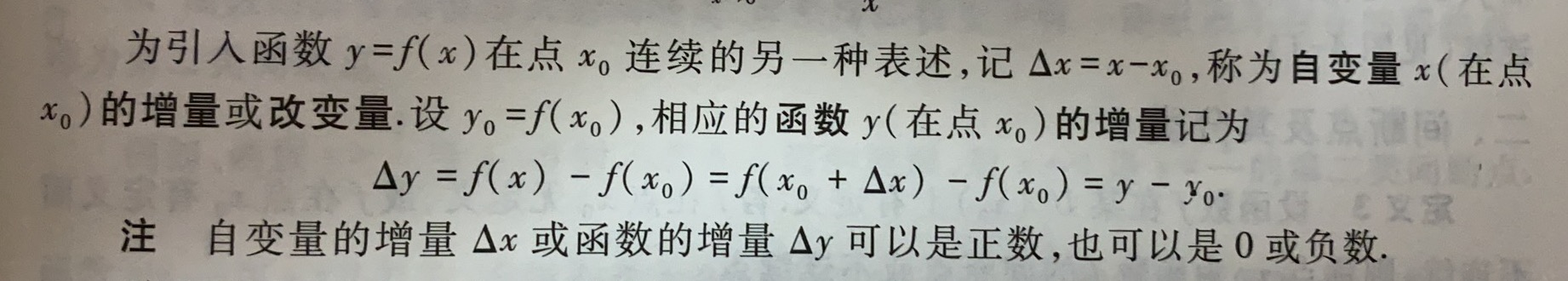(2)连续性的定义(3种):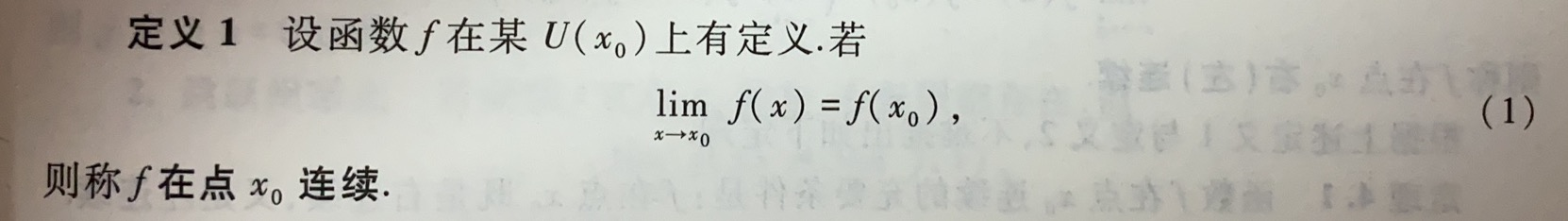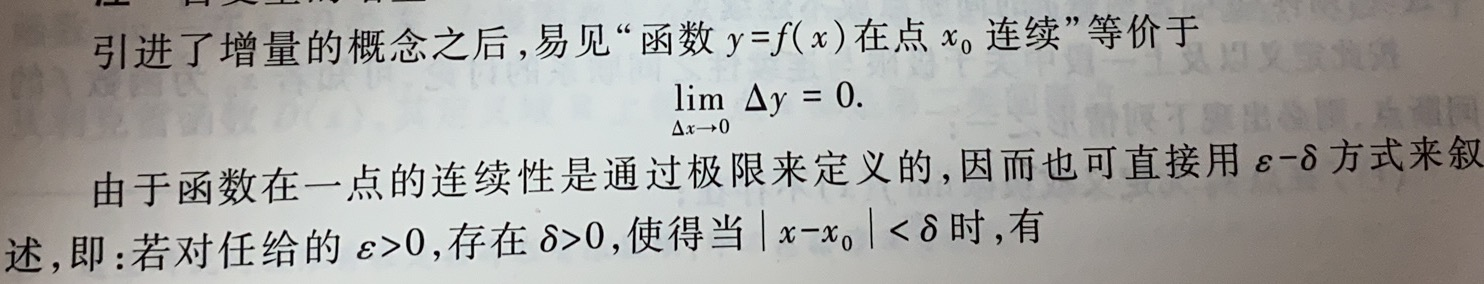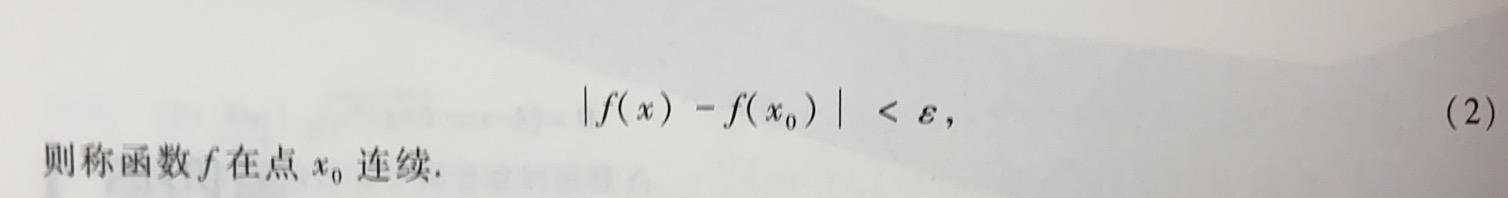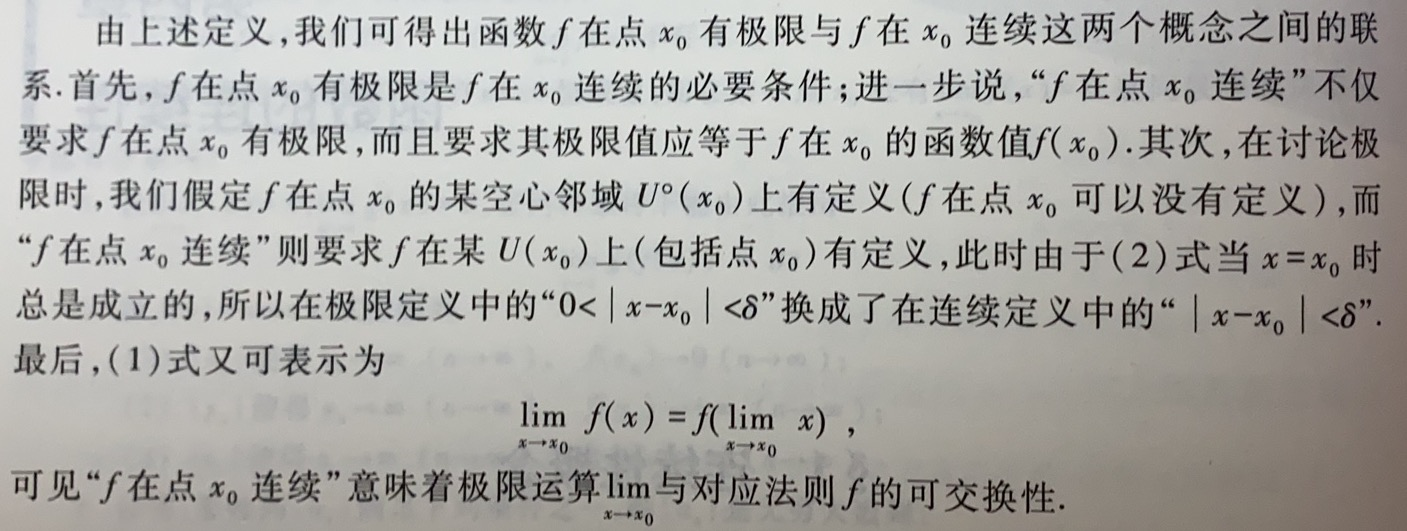2.左(右)连续性: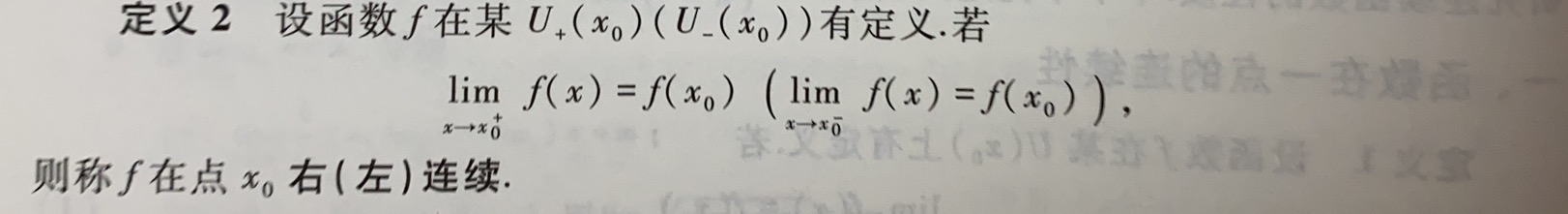3.函数连续的充要条件(定理4.1):

函数f在点x0处连续的充要条件是:f在x0处既是左连续的,又是右连续的

4.间断点 (1)定义:(2)分类:

函数f的间断点x0的情况必为下述3种之一:: ①f在x0处无定义 ②f在x0处有定义但

lim

⁡

x

→

x

0

f

(

x

)

\displaystyle \lim_{x \to x_0}{f(x)}

不存在 ③f在x0处有定义且

lim

⁡

x

→

x

0

f

(

x

)

\displaystyle \lim_{x \to x_0}{f(x)}

存在(指有限极限,不包括非正常极限),但

lim

⁡

x

→

x

0

f

(

x

)

\displaystyle \lim_{x \to x_0}{f(x)}

≠

f

(

x

0

)

f(x_0)

据此,可对函数的间断点进行分类

①第一类间断点:左/右极限均存在,仅包括以下2类 –i.可去间断点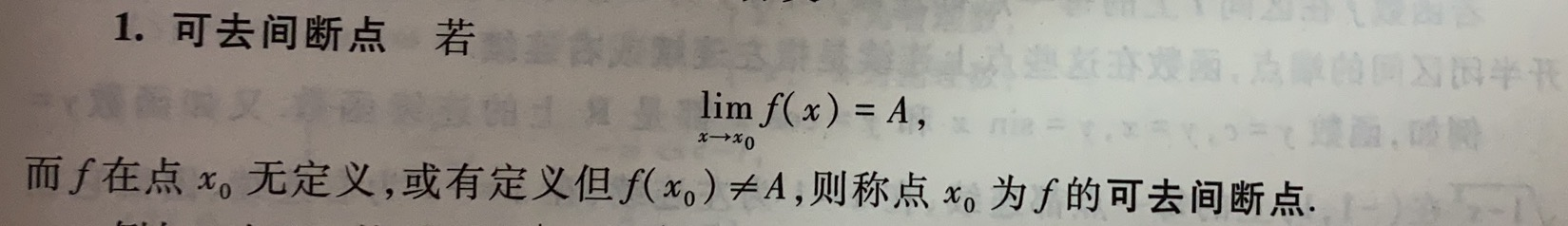可去间断点可通过下述方法转换成连续点: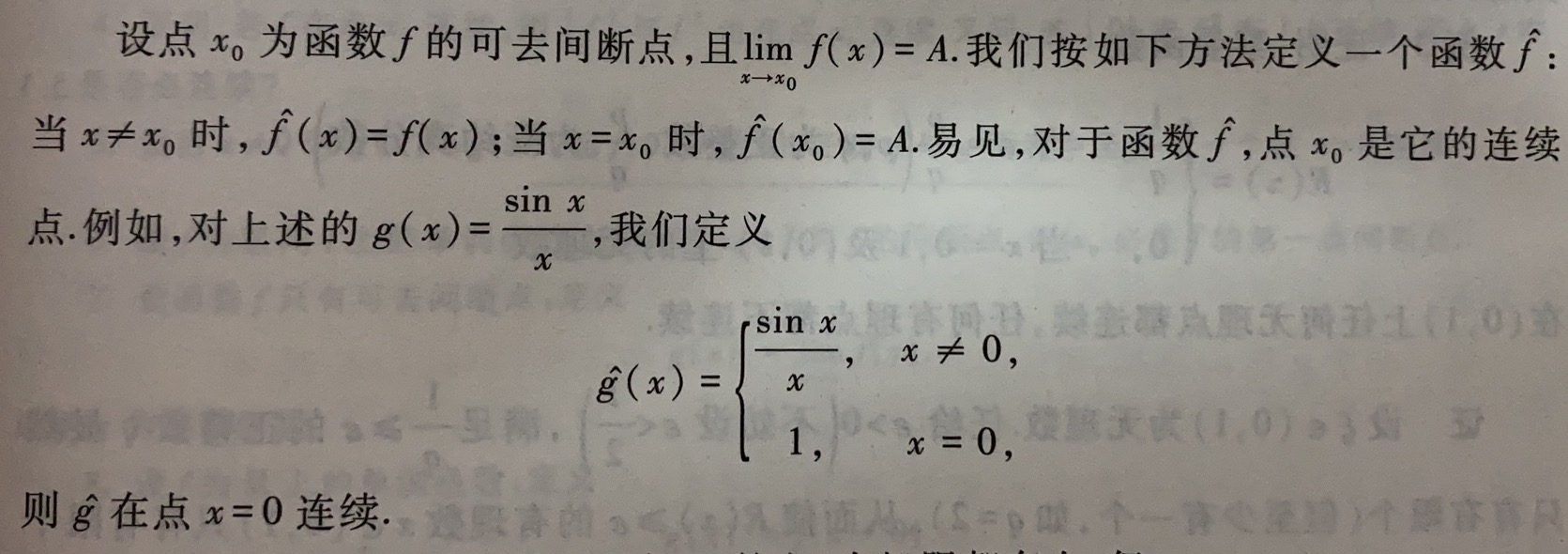–ii.跳跃间断点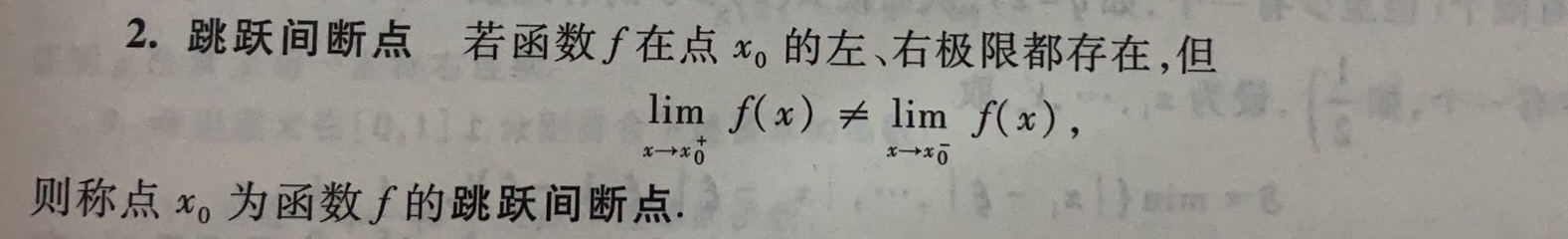第二类间断点:左/右极限至少有1个不存在(即其他形式的间断点),除以下2类还有很多类 –i.无穷间断点 –ii.震荡间断点

5.连续函数: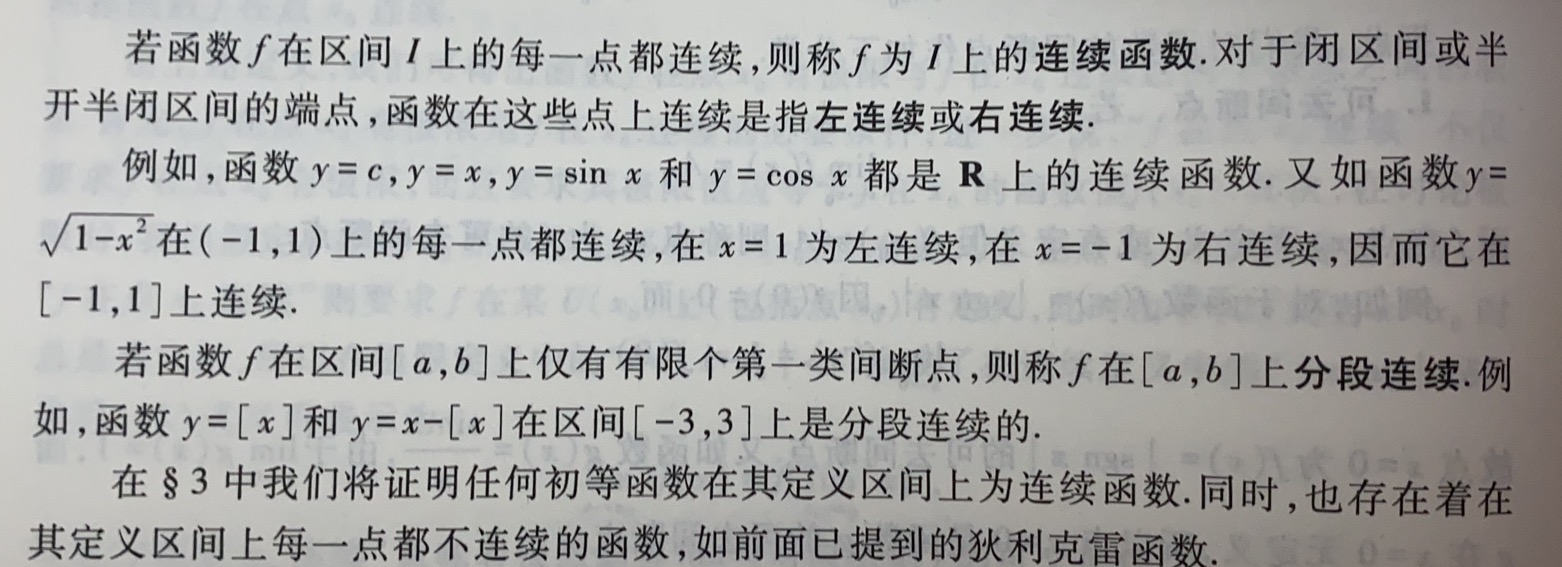二.连续函数的性质 1.连续函数的局部性质 (1)局部有界性(定理4.2):

若函数f在点x0处连续,则f在某U(x0)上有界

(2)局部保号性(定理4.3):

若函数f在点x0处连续,且f(x)>0(或<0),则对∀0<r<f(x0)(或0<r<-f(x0),∃某U(x0),使对∀x∈U(x0),有f(x)>r(或f(x)<-r) 在具体应用局部保号性时,常取r=

1

2

f

(

x

0

)

\frac{1}{2}f(x_0)

,则当

f

(

x

0

)

>

0

f(x_0)>0

时,∃某U(x0),使在其上有f(x)>

1

2

f

(

x

0

)

\frac{1}{2}f(x_0)

(3)有限次四则运算不改变连续性(定理4.4):

若函数f,g在点x0处连续,则

f

±

g

,

f

⋅

g

,

f

g

(

g

(

x

0

)

≠

0

)

f±g,f·g,\frac{f}{g}(g(x_0)≠0)

也都在x0处连续

(4)有限次复合运算不改变连续性(定理4.5):

若函数f在点x0处连续,g在点u0处连续,

u

0

=

f

(

x

0

)

u_0=f(x_0)

,则复合函数

g

○

f

g○f

在点x0处连续 根据连续性的定义,结论也可表示为

lim

⁡

x

→

x

0

g

(

f

(

x

)

)

=

g

(

lim

⁡

x

→

x

0

f

(

x

)

)

=

g

(

f

(

x

0

)

)

\displaystyle \lim_{x \to x_0}{g(f(x))}=g(\displaystyle \lim_{x \to x_0}{f(x)})=g(f(x_0))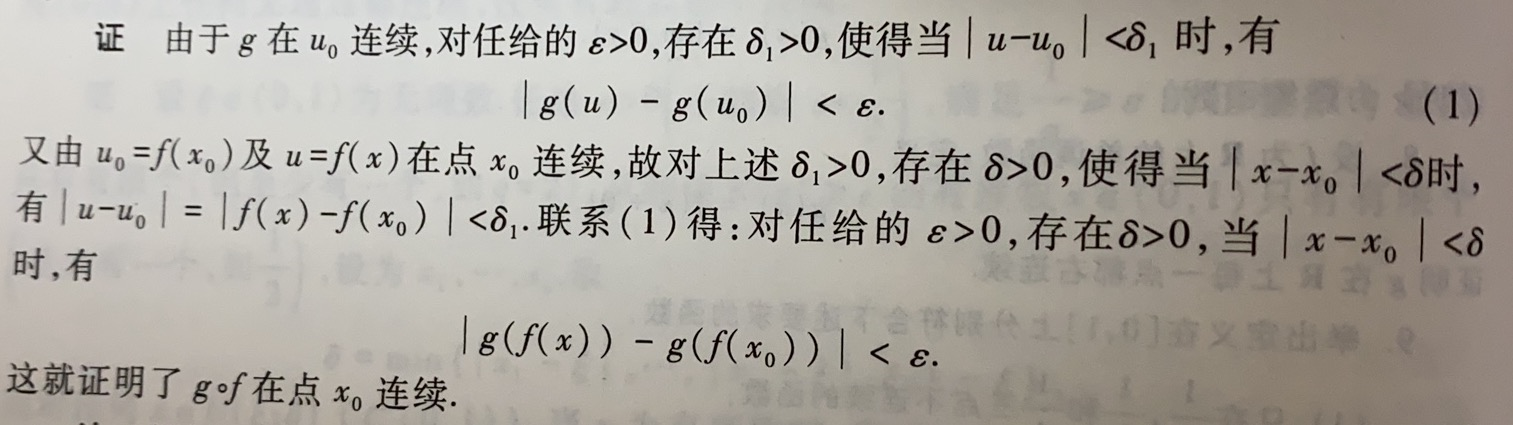扩展到可去间断点处: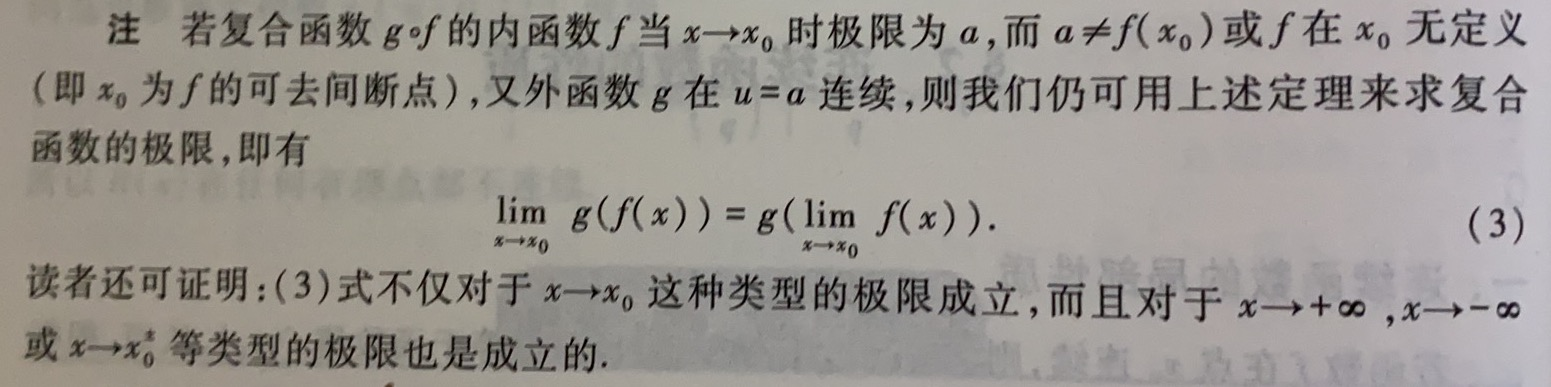2.闭区间上连续函数的性质 (1)最大值与最小值: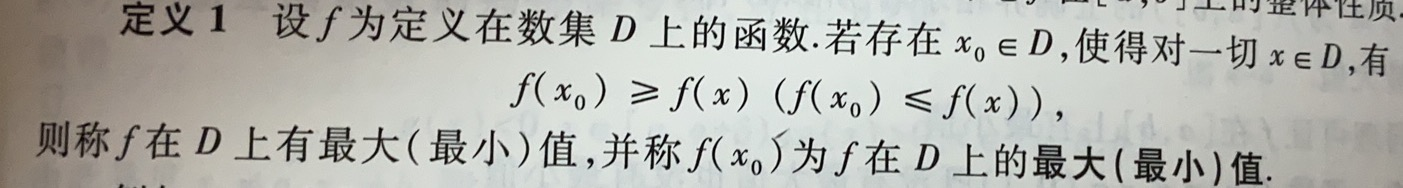(2)最大/最小值定理(定理4.6):

若函数f在闭区间[a,b]上连续,则f在[a,b]上有最大值和最小值

引理(有界性定理):若函数f(x)在闭区间[a,b]上连续,则f(x)在[a,b]上有界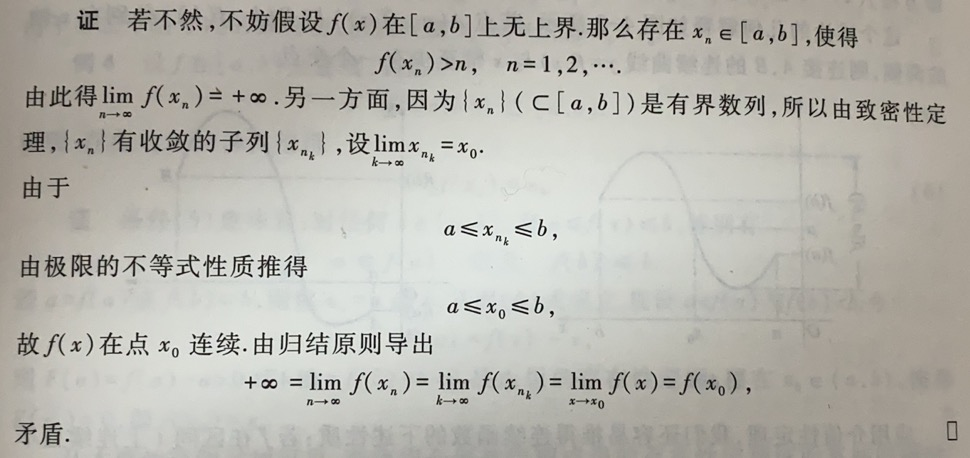定理4.6的证明: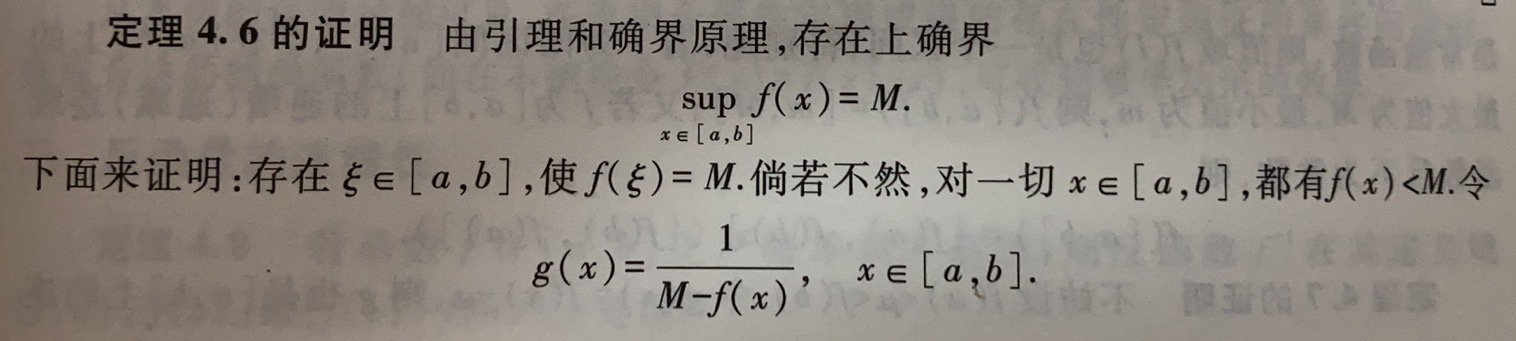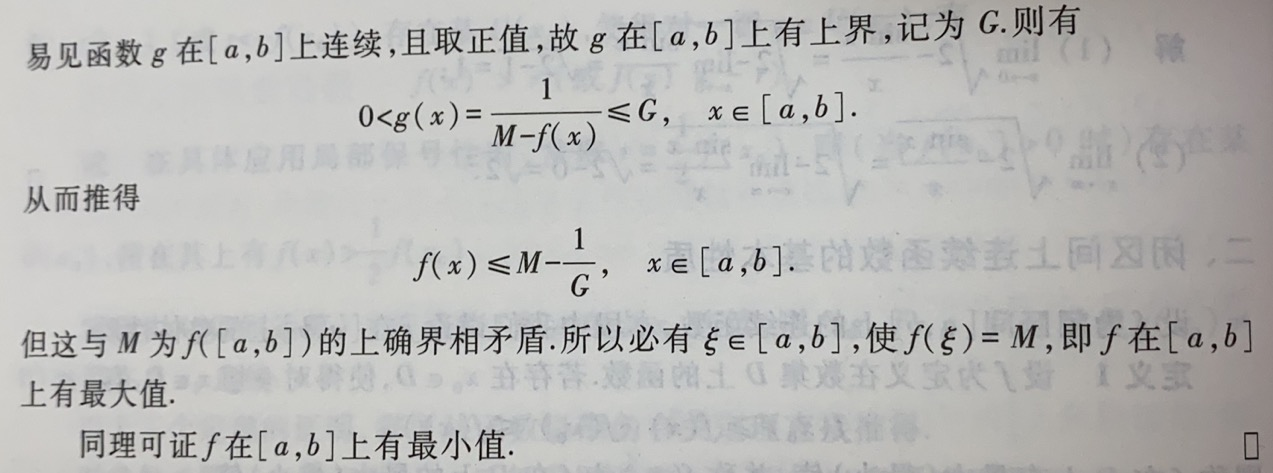(3)介值性定理(定理4.7):

设函数f在闭区间[a,b]上连续,且f(a)≠f(b),若μ为介于f(a)与f(b)间的任何实数(f(a)<μ<f(b)或f(a)>μ>f(b)),则至少∃1点x0∈(a,b),使f(x0)=μ 这个定理表面:若f在[a,b]上连续,不妨设f(a)<f(b),则f在[a,b]上必能取得区间[f(a),f(b)]上的一切值,即[f(a),f(b)]⫋f([a,b]) 该命题的几何意义如图4-2;下面的推论是定理4.7的等价命题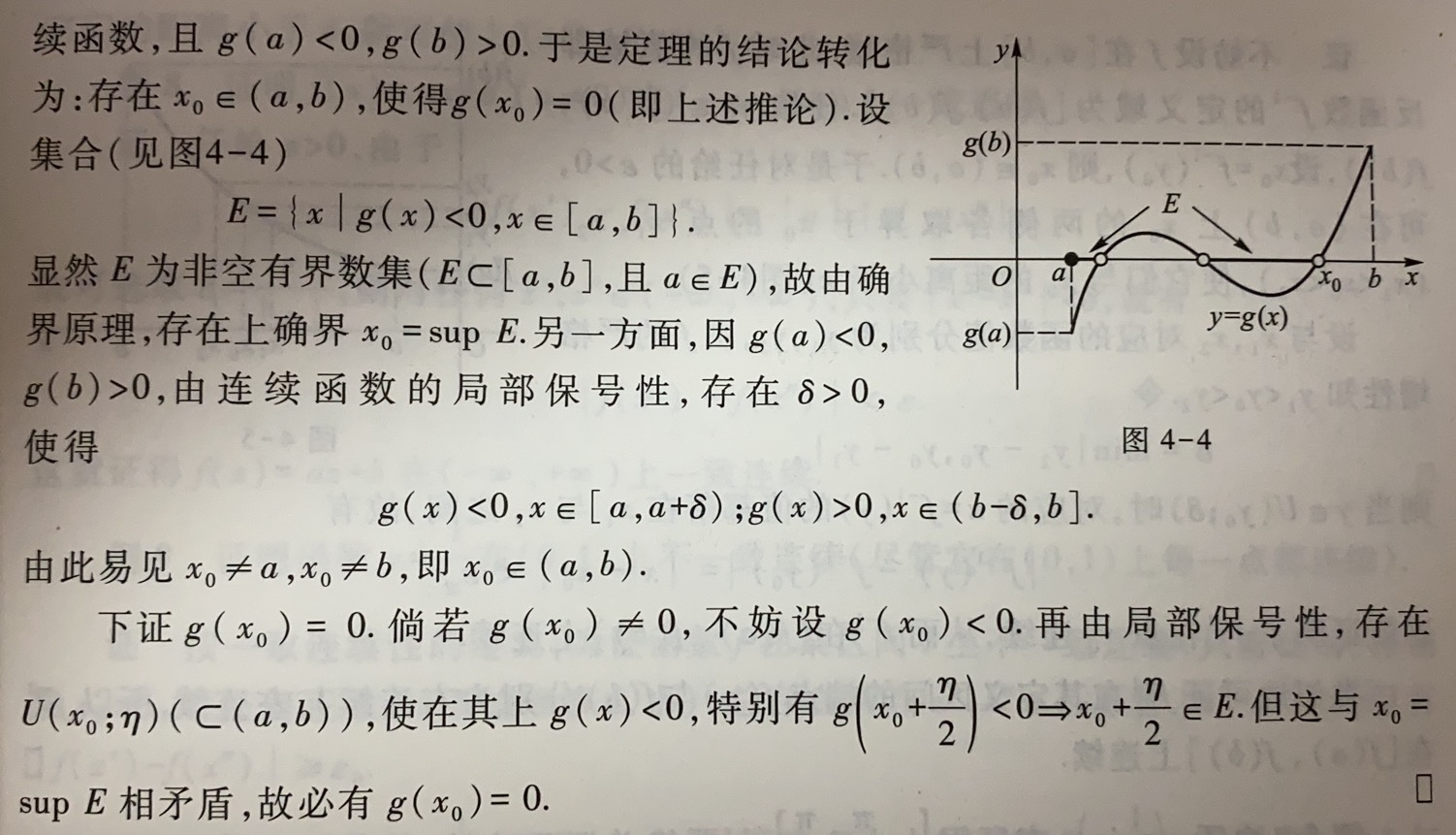推论(根的存在定理):若函数f在闭区间[a,b]上连续,且f(a)·f(b)<0,则至少∃1点x0∈(a,b),使f(x0)=0,即方程f(x)=0在(a,b)上至少有1个根 这个推论的几何解释如图4.3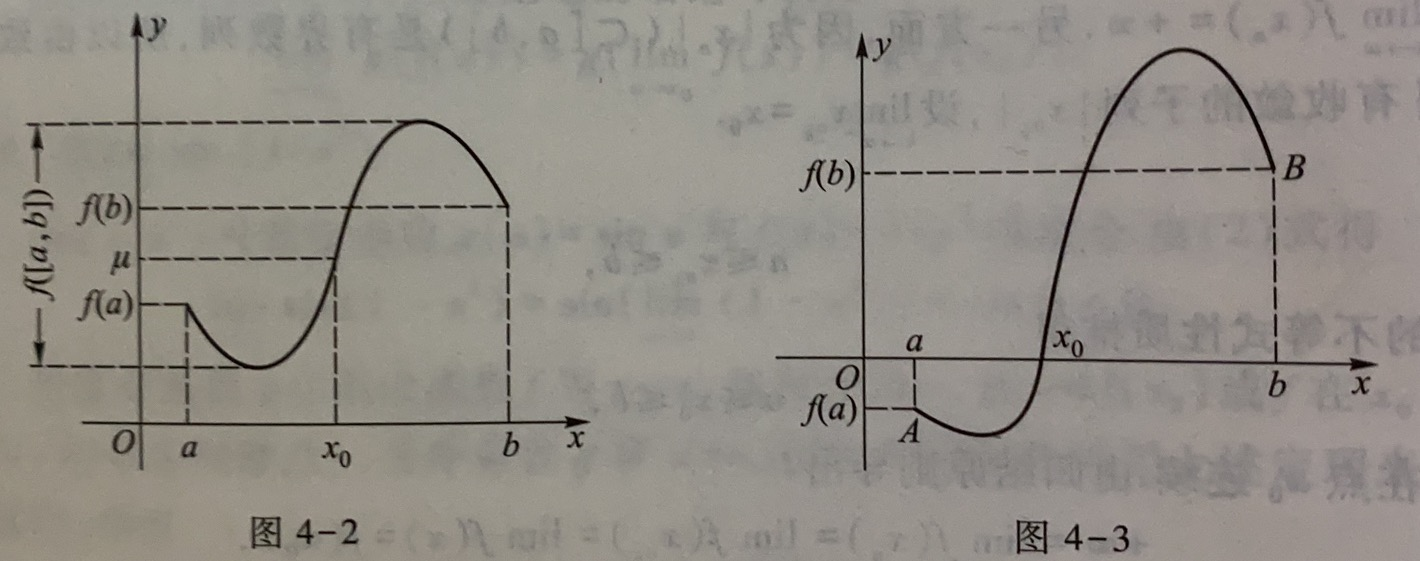由定理4.7可得性质:若f在区间I上连续且不为常量,则值域f(I)也是1个区间 特别地,若I为闭区间[a,b],f在[a,b]上的最大值/最小值为M/m,则f([a,b])=[m,M] 又若f为[a,b]上的递增(或减)连续函数且不为常函数,则f([a,b])=[f(a),f(b)] (或[f(b),f(a)])

3.反函数的连续性

定理4.8:若f在[a,b]上严格单调并连续,则其反函数f-1在其定义域[f(a),f(b)] (或[f(b),f(a)])上连续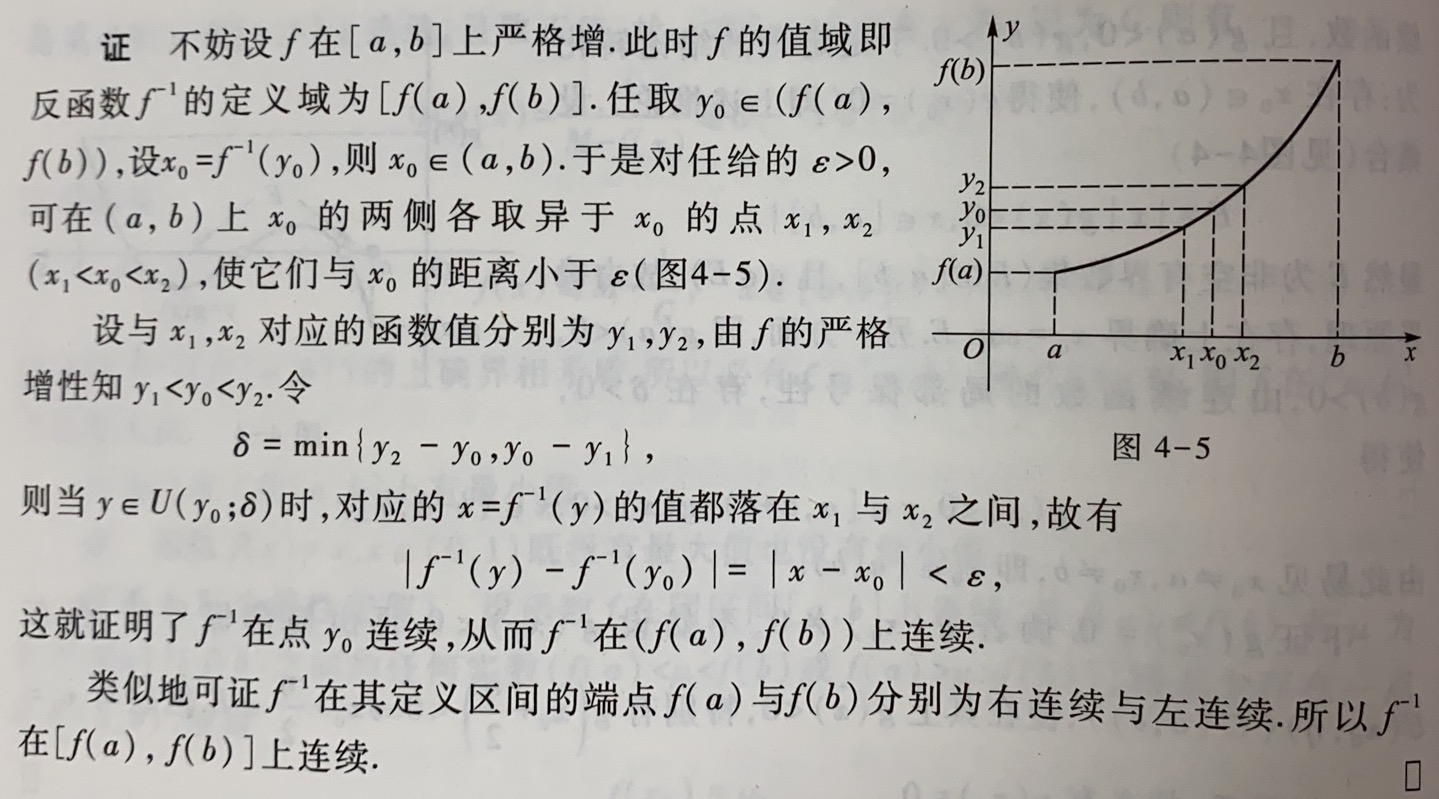三.一致连续性 1.定义: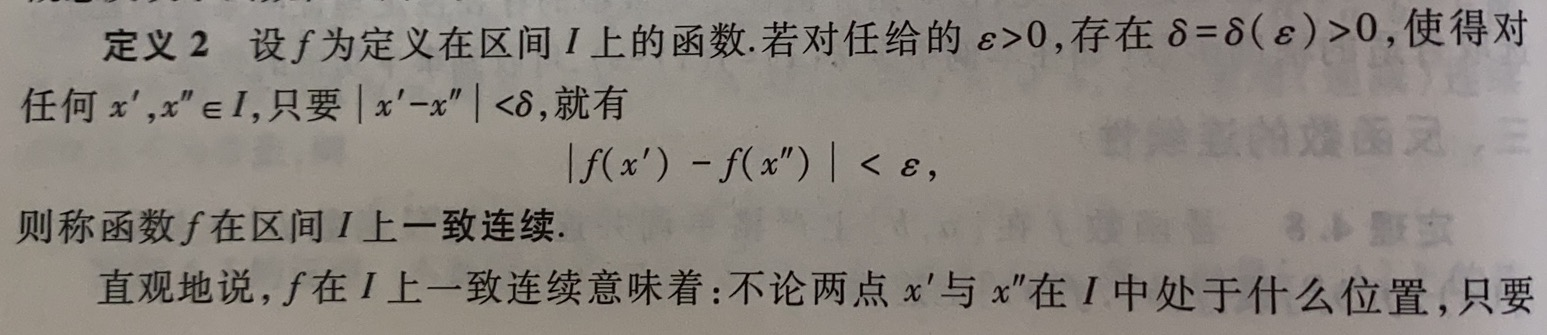2.一致连续性与连续性:

比如y=

1

x

\frac{1}{x}

在(0,1]上连续但不一致连续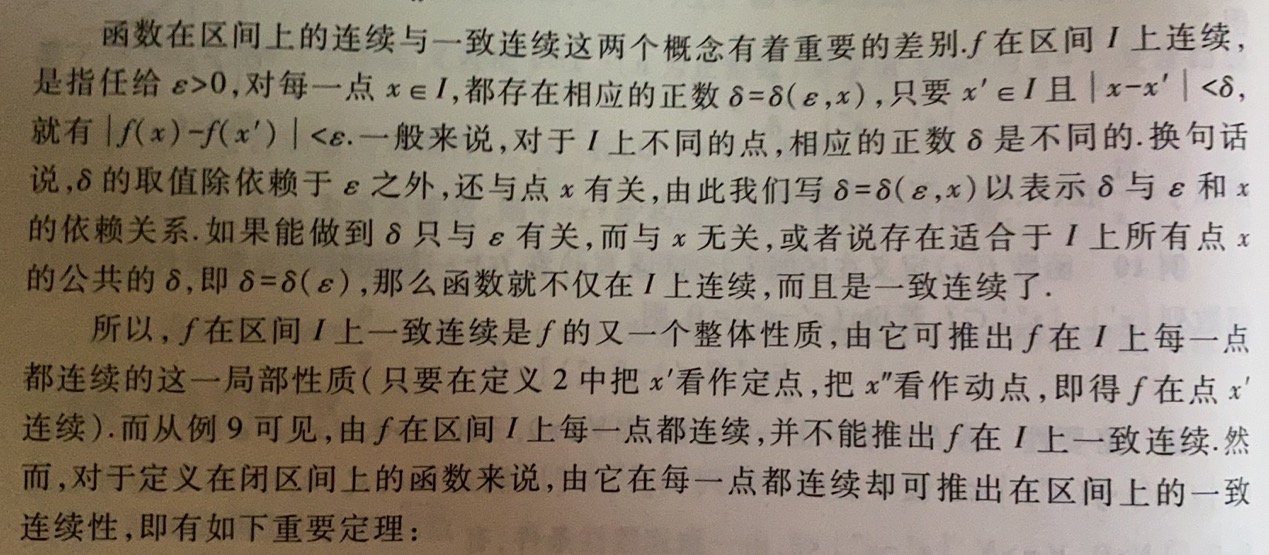3.一致连续性定理(定理4.9):

若f在闭区间[a,b]上连续,则f在[a,b]上一直连续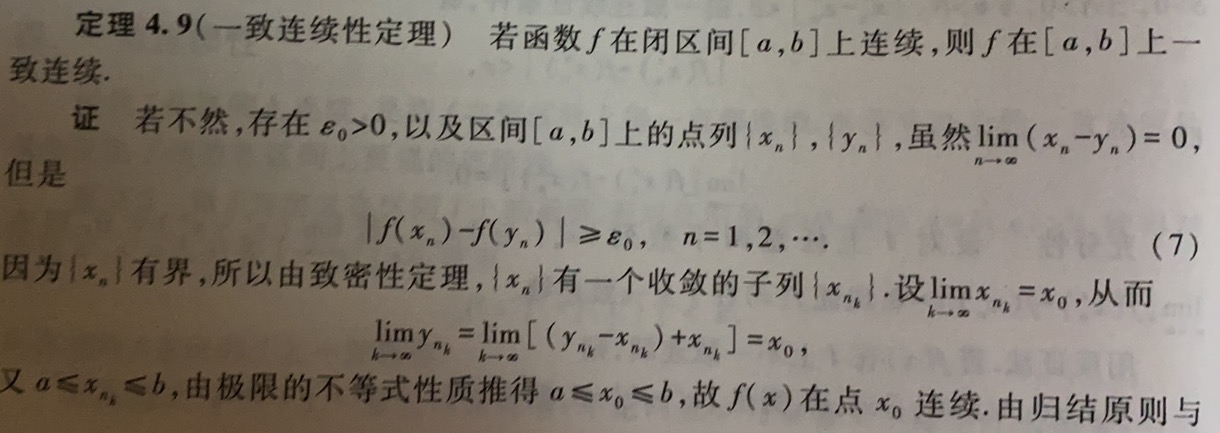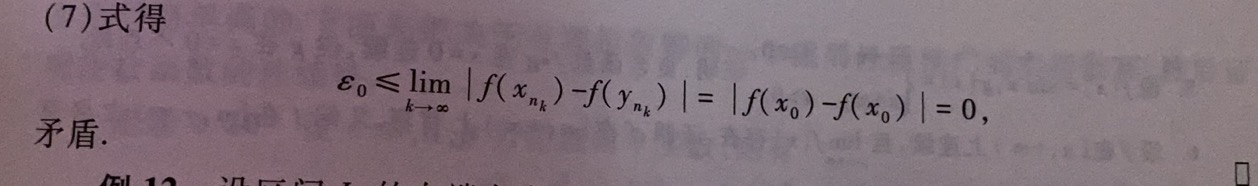4.任意区间上一致连续性的充要条件:

若f(x)定义在区间I上,f(x)在I上一致连续的充要条件是:对∀数列{x’n},{x’'n}⫋I,若

lim

⁡

n

→

∞

(

x

n

′

−

x

n

′

′

)

=

0

\displaystyle \lim_{n \to \infty}{(x'_n-x''_n)}=0

,则

lim

⁡

n

→

∞

[

f

(

x

n

′

)

−

f

(

x

n

′

′

)

]

=

0

\displaystyle \lim_{n \to \infty}{[f(x'_n)-f(x''_n)]}=0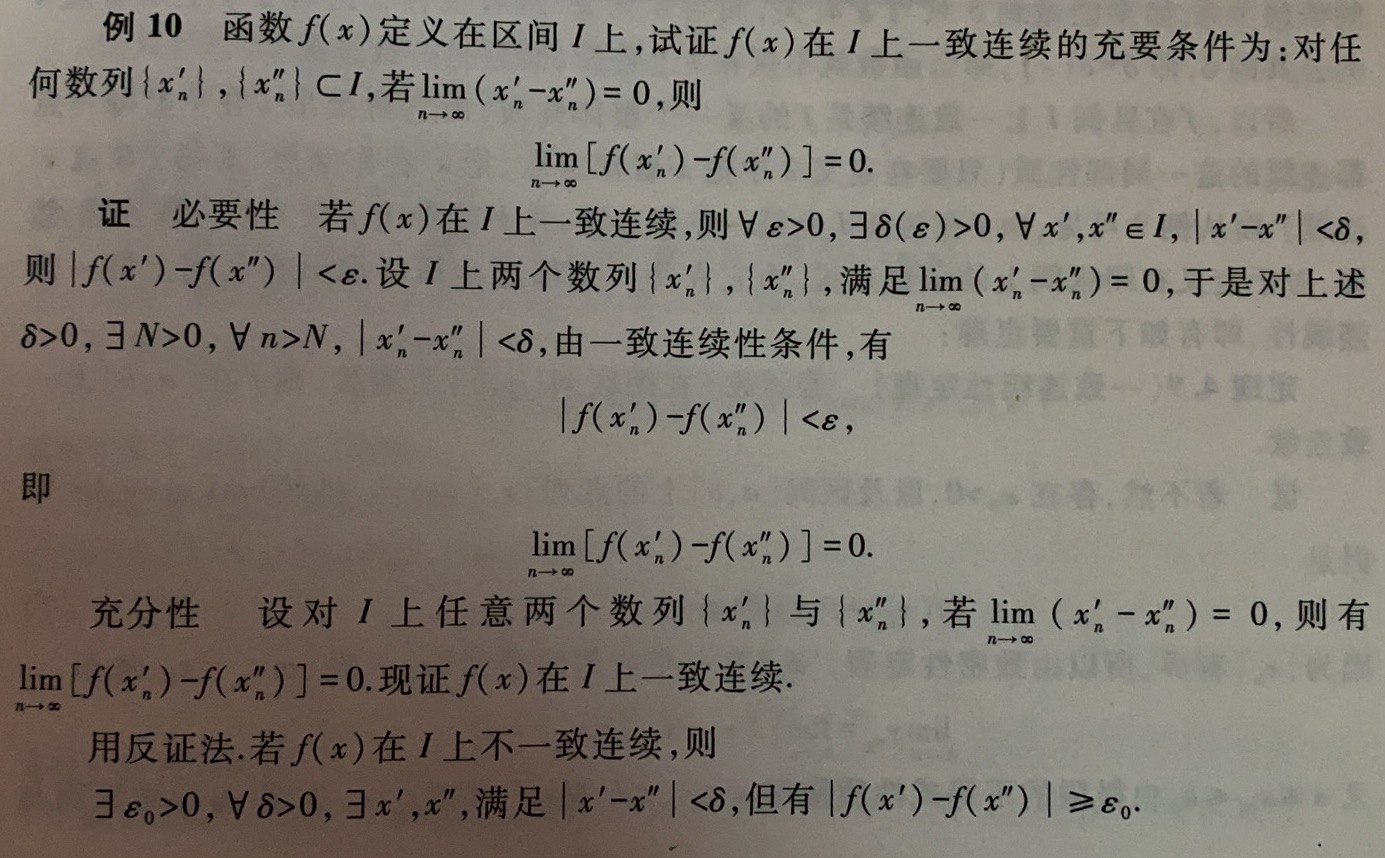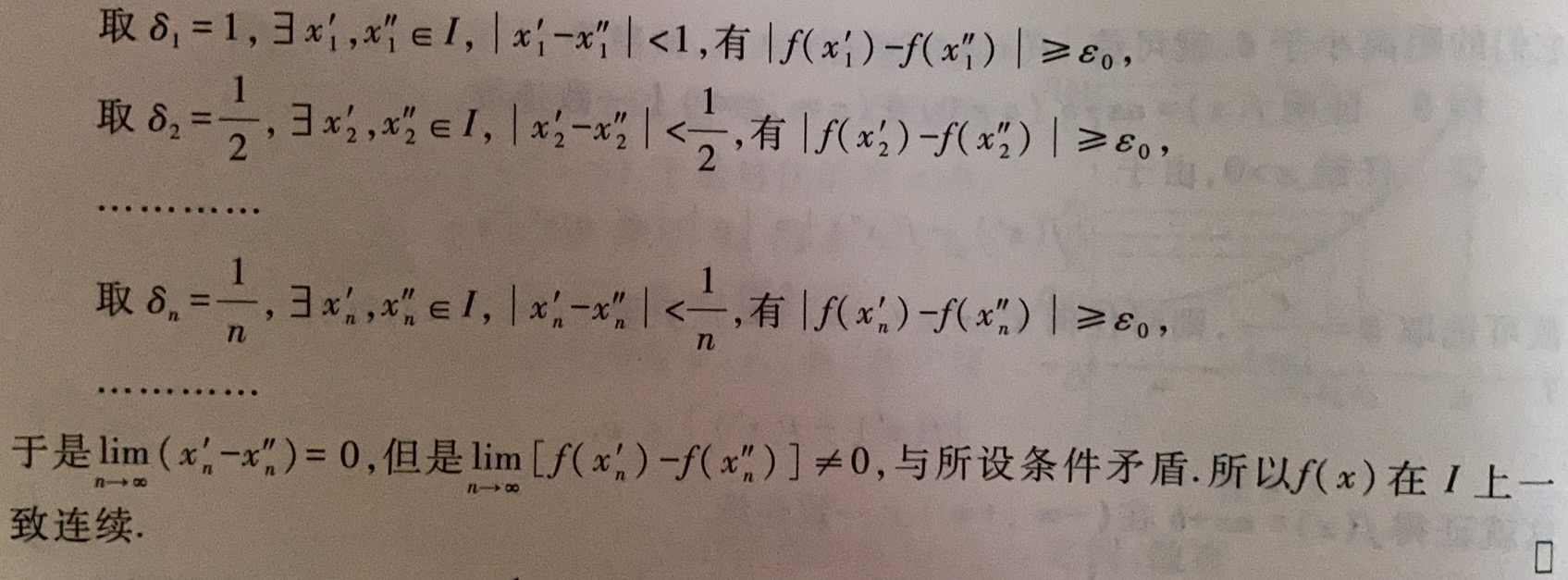如:

f

(

x

)

=

1

x

(

0

<

x

≤

1

)

f(x)=\frac{1}{x}(0<x≤1)

,取

x

n

′

=

1

n

n

≤

1

x'_n=\frac{1}{n^n}≤1

,

x

n

′

′

=

1

n

≤

1

x''_n=\frac{1}{n}≤1

,则

lim

⁡

n

→

∞

(

x

n

′

−

x

n

′

′

)

=

lim

⁡

n

→

∞

1

n

n

−

lim

⁡

n

→

∞

1

n

=

0

\displaystyle \lim_{n \to \infty}{(x'_n-x''_n)}=\displaystyle \lim_{n \to \infty}{\frac{1}{n^n}}-\displaystyle \lim_{n \to \infty}{\frac{1}{n}}=0

,但

lim

⁡

n

→

∞

[

f

(

x

n

′

)

−

f

(

x

n

′

′

)

]

=

lim

⁡

n

→

∞

n

n

−

lim

⁡

n

→

∞

n

=

+

∞

≠

0

\displaystyle \lim_{n \to \infty}{[f(x'_n)-f(x''_n)]}=\displaystyle \lim_{n \to \infty}{n^n}-\displaystyle \lim_{n \to \infty}{n}=+\infty≠0

,故

f

(

x

)

=

1

x

f(x)=\frac{1}{x}

在(0,1]上不一致连续

四.初等函数的连续性 1.初等函数的连续性 (1)基本初等函数的连续性(定理4.12):

一切基本初等函数都是其定义域上的连续函数

(2)初等函数的连续性(定理4.13):

任何初等函数都是其定义区间上的连续函数

2.证明 (1)三角函数连续性: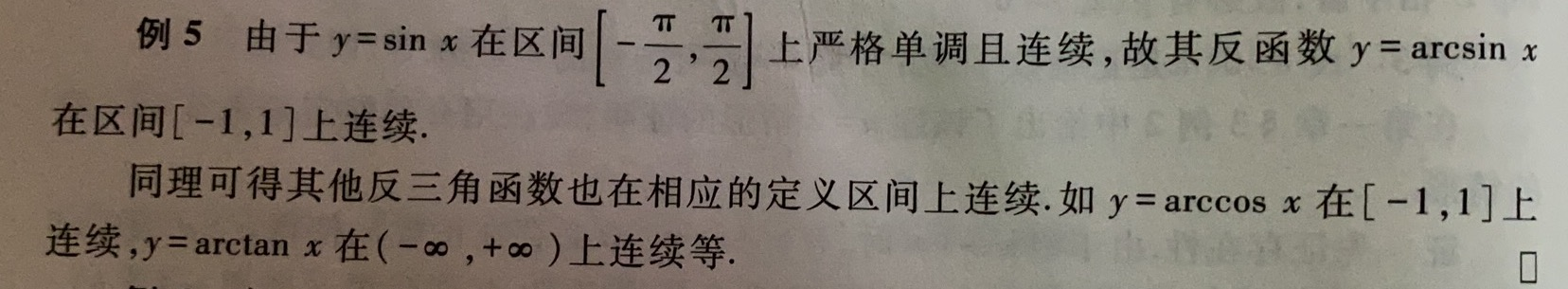(2)反三角函数的连续性:(3)幂函数:

–i.对多项式函数: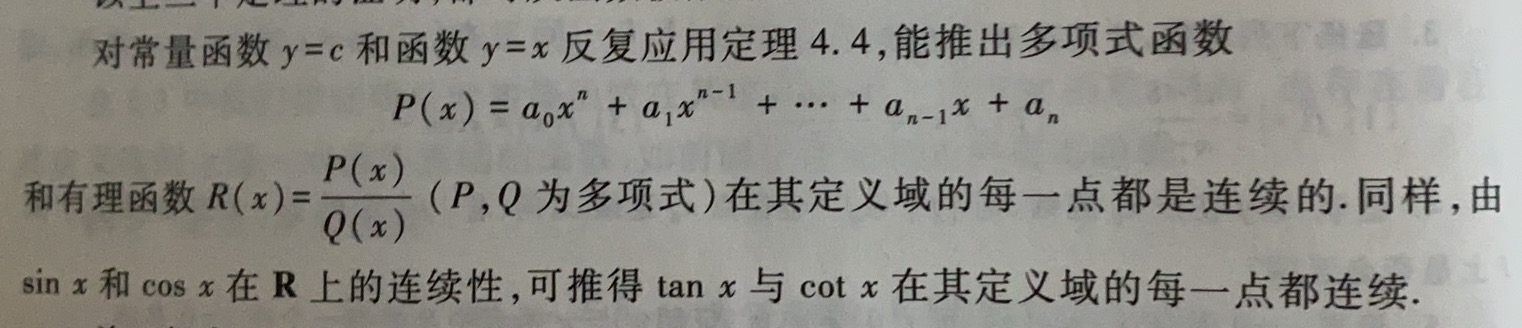–ii.对具有整数次幂的幂函数: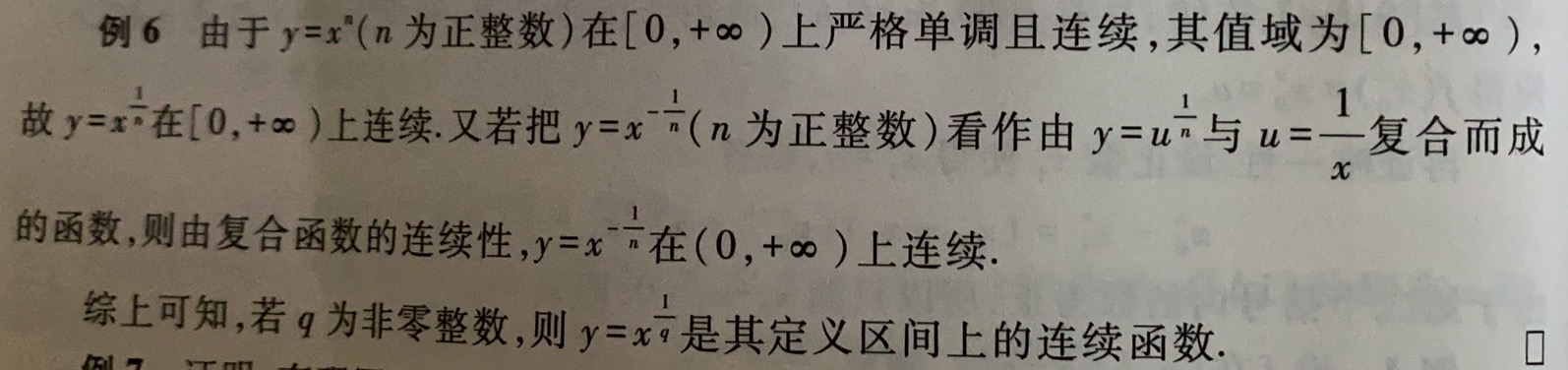–iii.对具有有理数次幂的幂函数: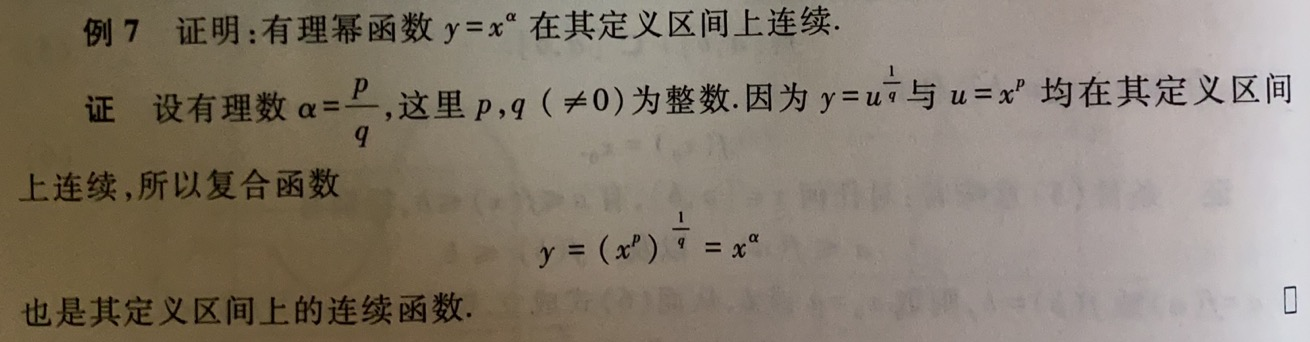–iv.对具有实数次幂的幂函数: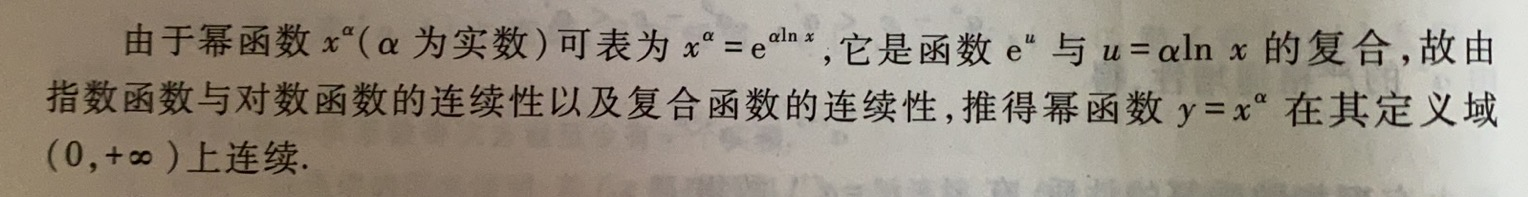(4)指数函数:

–i.定理4.10:设a>0,α,β为∀2个实数,则有aα·aβ=aα+β,(aα)β=aαβ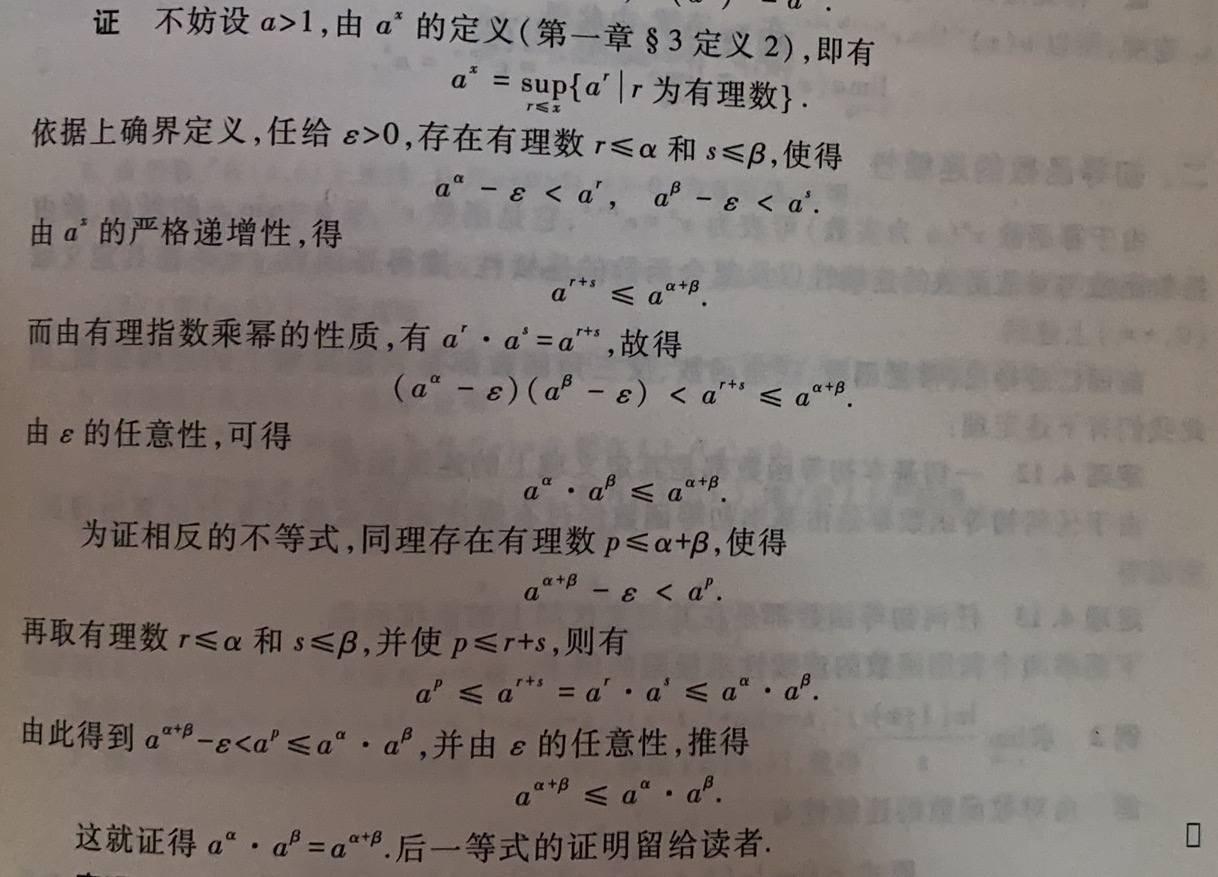–ii.定理4.11:指数函数ax(a>0)在R上是连续的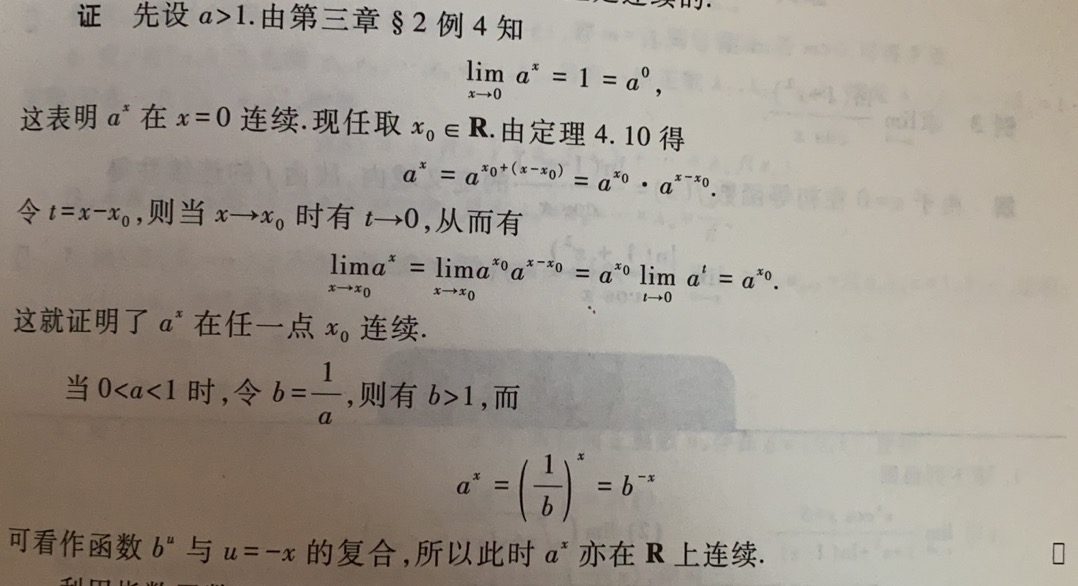(5)对数函数: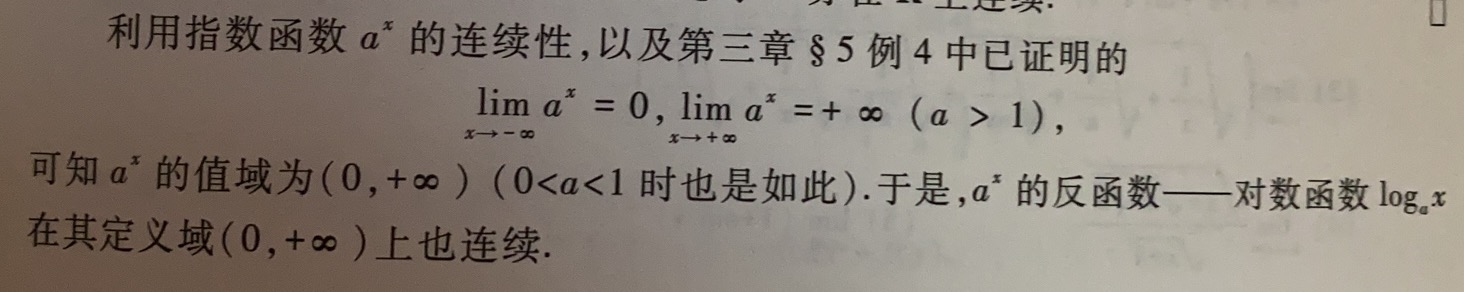展开全文• 关于连续函数列的极限函数的连续条件,最常见的是一致收敛的充分条件。后来狄尼又引入了较弱的广义一致收敛的概念,它仍然是一个充分而不必的条件。最后由阿尔捷拉给出了理想的准一致收敛的充分必要的条件。在中我们...
• 研究了具有阶段结构和有限时滞捕食一食饵系统的一致持久，并利用建立Lyapunov函数的方法得到了系统全局渐近稳定的充分条件
• 文章目录可鸡的第一充要条件证明必要性充分性可鸡的第一充要条件(可鸡准则)证明必要性充分性可鸡的第三充要条件证明必要性充分性两个题题①证明法①可鸡第二充要条件法②可鸡第三充要条件题②证明 证明函数可鸡的...


文章目录
可鸡的第一充要条件证明必要性充分性

可鸡的第二充要条件(可鸡准则)证明必要性充分性

可鸡的第三充要条件证明必要性充分性

两个题题①证明法①可鸡第二充要条件法②可鸡第三充要条件

题②证明

证明函数可鸡的道路总是那么艰辛，不过还好，总有一群机智的天才们想出了各种各样的定理来解决这个问题，这三个充要条件实在是太牛逼了，尤其是第三个，看起来奇奇怪怪的，但没想到用的还挺多？！每一个定理的证明都很牛逼，简洁清晰，但自己当时就是想不出来，我甚至还去百度了这三个充要条件到底是谁提出来的，然鹅，我没查到(￣ー￣)。。。
下面就介绍一下这三个充要条件以及它们的证明吧，这么优秀的定理我不允许有人不知道！！都给我看！！可鸡的第一充要条件

f

f

在

[

a

,

b

]

[a,b]

可鸡

⇔

\Leftrightarrow

S

=

s

S=s

证明
必要性

f

f

可鸡

⇒

S

=

s

?

\Rightarrow S=s?

令

J

=

∫

a

b

f

(

x

)

d

x

,

由

于

f

可

鸡

⇒

∀

ε

&gt;

0

,

∃

δ

&gt;

0

,

只

要

∣

∣

T

∣

∣

&lt;

δ

J=\int_a^bf(x)dx,由于f可鸡\Rightarrow\forall\varepsilon&gt;0,\exist\delta&gt;0,只要||T||&lt;\delta

∣

∑

i

=

1

n

f

(

ξ

i

)

△

x

i

−

J

∣

&lt;

ε

\Big|\sum\limits_{i=1}^nf(\xi_i)\triangle x_i-J\Big|&lt;\varepsilon

这说明只有分割满足模长条件，不管这个分割里的

ξ

\xi

取的是啥都OK，所以！

S

(

T

)

,

s

(

T

)

S(T),s(T)

也满足这个式子，因为他们只是相对于点集{

ξ

i

\xi_i

}的积分和而已

⇒

\Rightarrow

∣

S

(

T

)

−

J

∣

≤

ε

,

∣

s

(

T

)

−

J

∣

≤

ε

|S(T)-J|\le\varepsilon,|s(T)-J|\le\varepsilon

故当

∥

T

∥

→

0

\|T\|\to0

时，

S

(

T

)

→

J

,

s

(

T

)

→

J

S(T)\to J,s(T)\to J

又由达布定理

⇒

lim

⁡

∥

T

∥

→

0

S

(

T

)

=

S

,

lim

⁡

∥

T

∥

→

0

s

(

T

)

=

s

⇒

\Rightarrow \lim\limits_{\|T\|\to0}S(T)=S,\lim\limits_{\|T\|\to0}s(T)=s\Rightarrow

S

=

s

=

J

S=s=J

充分性

S

=

s

=

J

⇒

S=s=J\Rightarrow

lim

⁡

∥

T

∥

→

0

S

(

T

)

=

lim

⁡

∥

T

∥

→

0

s

(

T

)

=

J

\lim\limits_{\|T\|\to0}S(T)=\lim\limits_{\|T\|\to0}s(T)=J

⇒

∀

ε

&gt;

0

,

∃

δ

&gt;

0

,

当

∥

T

∥

&lt;

δ

,

有

\Rightarrow \forall\varepsilon&gt;0,\exist\delta&gt;0,当\|T\|&lt;\delta,有

J

−

ε

&lt;

s

(

T

)

≤

∑

i

=

1

n

f

(

ξ

i

)

△

x

i

≤

S

(

T

)

&lt;

S

(

T

)

+

ε

J-\varepsilon&lt;s(T)\le \sum_{i=1}^nf(\xi_i)\triangle x_i\le S(T)&lt;S(T)+\varepsilon

⇒

f

(

x

)

\Rightarrow f(x)

可鸡+

∫

a

b

f

(

x

)

d

x

=

J

\int_a^bf(x)dx=J

这个定理可以用来快速判断一个函数可鸡不，之前不是说了狄屎函数不可鸡嘛？就是因为它的上和(=1)和下和(=0)不相等
可鸡的第二充要条件(可鸡准则)
这是之前提到的唯一一个可鸡的充要条件，见函数可鸡条件，但是没有给出证明，在这里证明了可鸡第一充要条件以后补上了。

f

f

在

[

a

,

b

]

[a,b]

可鸡

⇔

\Leftrightarrow

∀

ε

&gt;

0

,

∃

T

⇒

S

(

T

)

−

s

(

T

)

&lt;

ε

\forall\varepsilon&gt;0,\exist T\Rightarrow S(T)-s(T)&lt;\varepsilon

即

∑

i

=

1

n

ω

i

△

x

i

&lt;

ε

即\sum\limits_{i=1}^{n}\omega_i\triangle x_i&lt;\varepsilon

证明
必要性

f

f

可鸡，由可鸡的第一充要条件

S

=

s

⇒

lim

⁡

∥

T

∥

→

0

[

S

(

T

)

−

s

(

T

)

]

=

0

S=s\Rightarrow\lim\limits_{\|T\|\to0}[S(T)-s(T)]=0

⇒

∀

ε

&gt;

0

,

∃

足

够

小

的

T

⇒

S

(

T

)

−

s

(

T

)

&lt;

ε

\Rightarrow\forall\varepsilon&gt;0,\exist 足够小的T\Rightarrow S(T)-s(T)&lt;\varepsilon

充分性
由于

s

(

T

)

≤

s

≤

S

≤

S

(

T

)

⇒

s(T)\le s\le S\le S(T)\Rightarrow

0

≤

S

−

s

≤

S

(

T

)

−

s

(

T

)

&lt;

ε

0\le S-s\le S(T)-s(T)&lt;\varepsilon

⇒

S

=

s

⇒

可

鸡

\Rightarrow S=s\Rightarrow可鸡

可鸡的第三充要条件

f

f

在

[

a

,

b

]

[a,b]

可鸡

⇔

\Leftrightarrow

∀

ε

,

η

&gt;

0

,

∃

T

\forall\varepsilon,\eta&gt;0,\exist T

,使得属于

T

T

的所有小区间中，振幅

ω

k

′

≥

ε

\omega_{k&#x27;}\ge\varepsilon

所在的区间

△

k

′

\triangle_{k&#x27;}

的总长

∑

k

′

△

x

k

′

&lt;

η

\sum\limits_{k&#x27;}\triangle x_{k&#x27;}&lt;\eta

证明
必要性

f

f

可鸡

⇒

\Rightarrow

由可鸡第二充要条件，令

σ

=

ε

η

&gt;

0

\sigma=\varepsilon\eta&gt;0

，则

∃

\exists

一个分割

T

⇒

T\Rightarrow

∑

k

ω

k

△

x

k

&lt;

σ

\sum\limits_{k}\omega_k\triangle x_k&lt;\sigma

⇒

\Rightarrow

ε

∑

k

′

△

x

k

′

&lt;

∑

k

′

ω

k

′

△

x

k

′

≤

∑

k

ω

k

△

x

k

&lt;

σ

=

ε

η

\varepsilon\sum\limits_{k&#x27;}\triangle x_{k&#x27;}&lt;\sum\limits_{k&#x27;}\omega_{k&#x27;}\triangle x_{k&#x27;}\le \sum\limits_{k}\omega_k\triangle x_k&lt;\sigma=\varepsilon\eta

⇒

∑

k

′

△

x

k

′

&lt;

η

\Rightarrow\sum\limits_{k&#x27;}\triangle x_{k&#x27;}&lt;\eta

充分性
由假设，

∃

\exists

某一分割，使得

ω

k

′

≥

ε

\omega_{k&#x27;}\ge\varepsilon

的那些

△

x

k

′

\triangle x_{k&#x27;}

的总长

∑

k

′

△

x

k

′

&lt;

η

\sum\limits_{k&#x27;}\triangle x_{k&#x27;}&lt;\eta

。 设

T

T

中剩下的满足

ω

k

′

′

&lt;

ε

\omega_{k&#x27;&#x27;}&lt;\varepsilon

的小区间们为

△

x

k

′

′

\triangle x_{k&#x27;&#x27;}

只要证

∑

k

ω

k

△

x

k

=

∑

k

′

ω

k

′

△

x

k

′

+

∑

k

′

′

ω

k

′

′

△

x

k

′

′

\sum\limits_{k}\omega_k\triangle x_k=\sum\limits_{k&#x27;}\omega_{k&#x27;}\triangle x_{k&#x27;}+\sum\limits_{k&#x27;&#x27;}\omega_{k&#x27;&#x27;}\triangle x_{k&#x27;&#x27;}

&lt;

(

M

−

m

)

∑

k

′

△

x

k

′

+

ε

∑

k

′

′

△

x

k

′

′

&lt;(M-m)\sum\limits_{k&#x27;}\triangle x_{k&#x27;}+\varepsilon\sum\limits_{k&#x27;&#x27;}\triangle x_{k&#x27;&#x27;}

≤

(

M

−

m

)

η

+

ε

(

b

−

a

)

&lt;

ε

′

\le (M-m)\eta+\varepsilon(b-a)&lt;\varepsilon&#x27;

所以，只要令

η

&lt;

ε

′

2

(

M

−

m

)

,

ε

&lt;

ε

′

2

(

b

−

a

)

\eta&lt;\frac{\varepsilon&#x27;}{2(M-m)},\varepsilon&lt;\frac{\varepsilon&#x27;}{2(b-a)}

即可
两个题
题①
证明黎曼函数在

[

0

,

1

]

[0,1]

上可鸡，且定积分为0
证明
这里可用可鸡充要条件②，③来证明，可以比较用哪个定理证明过程更简洁
法①可鸡第二充要条件

∀

ε

&gt;

0

,

在

[

0

,

1

]

\forall\varepsilon&gt;0,在[0,1]

上使得

1

q

&gt;

ε

2

\frac{1}{q}&gt;\frac{\varepsilon}{2}

的有理点总是有限的(且

1

q

≤

1

2

\frac {1}{q}\le \frac {1}{2}

)假设有

k

k

个，记为

r

1

,

.

.

.

,

r

k

r_1,...,r_k

对

[

0

,

1

]

[0,1]

做分割

T

=

{

△

1

,

.

.

.

,

△

n

}

T=\{\triangle_1,...,\triangle_n\}

,记包含

r

1

,

.

.

.

,

r

k

r_1,...,r_k

的小区间为

{

△

i

′

∣

i

=

1

,

.

.

.

,

m

}

\{\triangle_i&#x27;|i=1,...,m\}

，剩余的记作

{

△

i

′

′

∣

i

=

1

,

.

.

.

,

n

−

m

}

\{\triangle_i&#x27;&#x27;|i=1,...,n-m\}

m

≤

2

k

m\le 2k

(只有所有

r

i

r_i

都是

T

T

的分割点时才有

m

=

2

k

m=2k

)在

△

i

′

\triangle_i&#x27;

上

f

f

的振幅

ω

i

′

≤

1

2

⇒

\omega_i&#x27;\le\frac {1}{2}\Rightarrow

∑

i

=

1

m

ω

i

′

△

x

i

′

≤

1

2

∑

i

=

1

m

△

x

i

′

≤

1

2

⋅

2

k

⋅

∥

T

∥

\sum\limits_{i=1}^{m}\omega_i&#x27;\triangle x_i&#x27;\le\frac{1}{2}\sum\limits_{i=1}^{m}\triangle x_i&#x27;\le \frac{1}{2}\cdot2k\cdot\|T\|

在

△

i

′

′

\triangle_i&#x27;&#x27;

上

f

f

的振幅

ω

i

′

′

≤

ε

2

⇒

\omega_i&#x27;&#x27;\le\frac {\varepsilon}{2}\Rightarrow

∑

i

=

1

n

−

m

ω

i

′

′

△

x

i

′

′

≤

ε

2

∑

i

=

1

n

−

m

△

x

i

′

′

&lt;

ε

2

\sum\limits_{i=1}^{n-m}\omega_i&#x27;&#x27;\triangle x_i&#x27;&#x27;\le\frac{\varepsilon}{2}\sum\limits_{i=1}^{n-m}\triangle x_i&#x27;&#x27;&lt;\frac{\varepsilon}{2}

So,只要

∥

T

∥

&lt;

ε

2

k

⇒

\|T\|&lt;\frac{\varepsilon}{2k}\Rightarrow

∑

i

=

1

n

ω

i

△

x

i

&lt;

ε

\sum\limits_{i=1}^{n}\omega_i\triangle x_i&lt;\varepsilon

证得

f

f

可鸡后，

J

=

∫

0

1

f

(

x

)

d

x

=

lim

⁡

∥

T

∥

→

0

∑

i

=

1

n

f

(

ξ

i

)

△

x

i

J=\int_0^1f(x)dx=\lim\limits_{\|T\|\to0}\sum\limits_{i=1}^{n}f(\xi_i)\triangle x_i

这里只要让所有的

ξ

i

\xi_i

取成无理数点即可

⇒

J

=

0

\Rightarrow J=0

法②可鸡第三充要条件
由法①得，含

1

q

≥

ε

\frac{1}{q}\ge\varepsilon

有理点的区间至多为

2

k

2k

个，在这些区间中有

ω

k

′

≥

ε

\omega_{k&#x27;}\ge\varepsilon

要证可鸡

⇒

∑

k

′

△

x

i

′

&lt;

η

⟺

\Rightarrow\sum\limits_{k&#x27;}\triangle x_i&#x27;&lt;\eta\Longleftrightarrow

∑

k

′

△

x

i

′

≤

2

k

∥

T

∥

&lt;

η

\sum\limits_{k&#x27;}\triangle x_i&#x27;\le2k\|T\|&lt;\eta

⇒

令

∥

T

∥

&lt;

η

2

k

即

可

\Rightarrow令\|T\|&lt;\frac{\eta}{2k}即可

由于

m

i

=

i

n

f

x

∈

△

i

f

(

x

)

=

0

⇒

s

(

T

)

≡

0

=

s

⇒

∫

0

1

f

(

x

)

d

x

=

s

=

0

m_i=\mathop{inf}\limits_{x\in\triangle_i}f(x)=0\Rightarrow s(T)\equiv0=s\Rightarrow \int_0^1f(x)dx=s=0

很明显，第三充要条件赢了，发现此时是通过区间个数有限来证明区间长度

&lt;

η

&lt;\eta

的
题②

f

f

在

[

a

,

b

]

[a,b]

上连续

φ

\varphi

在

[

a

,

b

]

[a,b]

上可鸡

a

≤

φ

(

t

)

≤

b

,

t

∈

[

α

,

β

]

a\le\varphi(t)\le b,t\in[\alpha,\beta]

⇒

f

∘

g

\Rightarrow f\circ g

在

[

α

,

β

]

[\alpha,\beta]

上可鸡
我就说之前关于定积分性质怎么没有关于复合函数的呢，原来在这里！
证明

f

f

在

[

a

,

b

]

[a,b]

上连续

⇒

\Rightarrow

一致连续

⇒

\Rightarrow

对

∀

ε

&gt;

0

,

∃

σ

&gt;

0

,

当

x

′

,

x

′

′

∈

[

a

,

b

]

满

足

∣

x

′

−

x

′

′

∣

&lt;

σ

时

，

对\forall\varepsilon&gt;0,\exist\sigma&gt;0,当x&#x27;,x&#x27;&#x27;\in[a,b]满足|x&#x27;-x&#x27;&#x27;|&lt;\sigma时，

∣

f

(

x

′

)

−

f

(

x

′

′

)

∣

&lt;

ε

|f(x&#x27;)-f(x&#x27;&#x27;)|&lt;\varepsilon

由于

φ

\varphi

在

[

α

,

β

]

[\alpha,\beta]

可鸡,由可鸡第三充要条件

⇒

∀

η

&gt;

0

,

∃

分

割

T

，

\Rightarrow\forall\eta&gt;0,\exist分割T，

使得在

T

T

中，有

ω

k

′

φ

≥

σ

\omega_{k&#x27;}^{\varphi}\ge\sigma

的小区间

△

k

′

\triangle_{k&#x27;}

长度之和

∑

k

′

△

k

′

&lt;

η

\sum\limits_{k&#x27;}\triangle_{k&#x27;}&lt;\eta

而在其他小区间

△

k

′

′

\triangle_{k&#x27;&#x27;}

中

ω

k

′

′

φ

&lt;

σ

\omega_{k&#x27;&#x27;}^{\varphi}&lt;\sigma

令

F

(

t

)

=

f

(

φ

(

t

)

)

,

t

∈

[

α

,

β

]

F(t)=f(\varphi(t)),t\in[\alpha,\beta]

⇒

\Rightarrow

在

T

T

的小区间

△

k

′

′

\triangle_{k&#x27;&#x27;}

上，

ω

k

′

′

F

&lt;

ε

\omega_{k&#x27;&#x27;}^{F}&lt;\varepsilon

⇒

\Rightarrow

至多在所有

△

k

′

\triangle_{k&#x27;}

上

ω

k

′

F

≥

ε

，

\omega_{k&#x27;}^{F}\ge\varepsilon，

但这些区间的长度之和至多为

∑

k

′

△

k

′

&lt;

η

\sum\limits_{k&#x27;}\triangle_{k&#x27;}&lt;\eta

由可鸡第三充要条件

⇒

f

∘

g

\Rightarrow f\circ g

可鸡
我发现！在可鸡证明中尤其要注意条件连续

⇒

\Rightarrow

一致连续。由一致连续神奇的性质可以与将它与区间内的振幅联系起来哦
展开全文• 连续性的概念 为了引入函数连续性的多种定义，我们记△x为x的增量 || 定义一：函数在一点上连续性定义（极限） 设函数f在某U(x0)内有定义，若limx–>x0f(x) = f(x0)，则称f在点x0处连续 || 定义一的另一种形式...
• 本文研究了通过采样控制实现双积分动力学的共识问题。 通过使用周期采样技术和零阶保持电路，从连续时间... 还获得了必要且充分条件，这与采样周期趋于零时的特殊情况相一致。 通过仿真证明了这些算法的有效
• 在给定任意的切换规则下, 运用多Lyapunov 函数和平均驻留时间方法设计使得闭环系统一致有限时间稳定和有限时间有界的状态反馈控制器, 同时给出基于线性矩阵不等式表示的控制器存在的充分条件. 最后通过数值算例表明...
• 分布式系统是一个非常有趣的话题, 在我之前的几年工作当中发现很多开发人员在评估和使用分布式系统的时候比较关注 benchmark 和高可用, 但经常不太关心分布式系统的一致性问题, 所以我打算在业余时间花点时间写...
• 在理解分析分布式一致性协议前，我们必须先看下CAP理论 CAP CAP是指在一个分布式系统中，一致性（Consistency）、可用（Availability）、分区容错（Partition tolerance）这三个要素最多只能同时实现两点，不...分布式 区块链 raft zookeeper
• 业务连续性管理峰会2007演讲记录2007-09-24 13:11 作者： 佚名 出处： 天极Yesky 责任编辑：黄惟冰 演讲一：从国际标准中看业务持续性管理的发展　申屠献忠 通标标准技术服务有限公司副总裁　我曾经参加很多经销商...项目管理 exchange microsoft security
• 分布式一致性与共识算法 UTXO 与账户余额模型 区块链技术是近几年逐渐变得非常热门的技术，以比特币为首的密码货币其实已经被无数人所知晓，但是却很少有人会去研究它们的底层技术，也就是作为一个分布式网络比特币...
• 研究了具有分布连续和离散时滞三个成长阶段的单种群模型,得到了与时滞有关的系统一致持久充分条件;同时通过构造 Lyapunov函数得到了与时滞有关的系统全局渐近稳定的充分条件。最后由已知的文献推导出系统在持久...
• 文章目录写在前面一致性算法连续时间离散时间一致性证明连续时间离散时间矩阵理论特征值和特征向量特征多项式代数重数几何重数总结 写在前面 最近在看一些分布式优化的文章，但是大部分文章都是用的离散时间算法。我...线性代数 矩阵
• 按照继承，可求，收敛原则定义[a，b]上几乎处处连续的本性函数的积分，引进一致局部可积与无穷断度点上积分一致收敛概念，给出函数可积的充要条件
• 特定 Mamda ni 模糊系统是指采用模糊单点作为规则后件的多输入单输出 Mamdani 模糊系 统。在每个输入变量的模糊子集满足一致性以及隶属函数连续且分段可微的条件下, 证明了特定 ...意连续实函数的充分条件。</p>多项式
• 基于矩阵指数和随机分析技术，给出了几个充分条件，以确保具有采样数据和数据包丢失的二阶多主体网络几乎可以肯定地同步，其中主体之间的图是有向网络。包含有向生成树。 另外，我们进一步研究了这种包含时延和...研究论文
• Raft 算法是可以用来替代 Paxos 算法的分布式一致性算法，而且 raft 算法比 Paxos 算法更易懂且更容易实现。本文对 raft 论文进行翻译，希望能有助于读者更方便地理解 raft 的思想。如果对 Paxos 算法感兴趣，可以...分布式 大数据
• 其“备份窗口”接近于零，可大大增加系统业务的连续性，为实现系统真正的7×24运转提供了保证。  三、 数据一致性  在容灾数据复制的应用种，能否保持数据的一致性是决定容灾效果的关键。我们从上文...oracle 数据备份 磁盘 数据库 存储系统
• 本文主要介绍TI C6000系列的C64x+的DSP Cache配置，常见的Cache miss模式以及如何在多核或者多外设DMA系统下维护Cache一致性，当然还有Cache优化的专题，针对指令L1P和数据L1D cache的优化。 C64x+与C64x CACHE...
• 研究一阶连续多智能体系统的一致性...通过分析多智能体系统的稳定,获得了一致性成立的充要条件,该条件揭示了交流拓扑、控制器增益、采样器的周期和保持器的周期的关系.最后,提供一个仿真例子以说明理论结果的有效.
• 研究紧致度量空间(X,d)上的连续自映射f,证明了若f具有等度连续性,则有:①f的链回归集与一致几乎周期点集相等,即CR(f)=UA(f),并举例说明了此结论不能进一步加强到CR(f)=P(f);②∩fn(X)=UA(f)。最后给出了f是等度连续......